## Getting Started with Nuvoton 8-bit Microcontrollers – Coding Part 3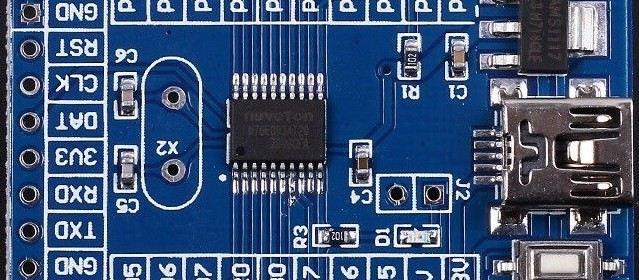This post is a continuation of the previous post on Nuvoton N76E003 microcontroller here.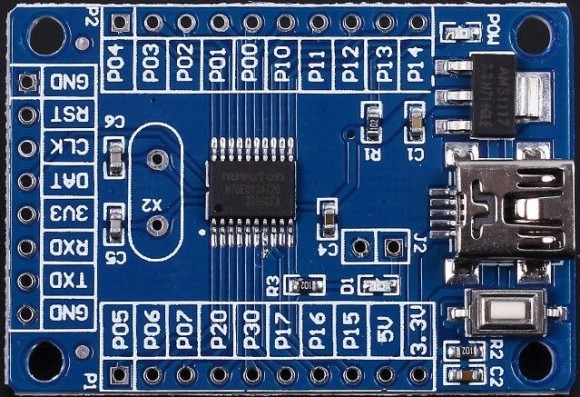# Communication Peripheral Overview

N76E003 packs basic serial communication peripherals for I2C, SPI and UART. These interfaces enable us to interface external EEPROM and flash memories, real-time clocks (RTC), sensors, etc.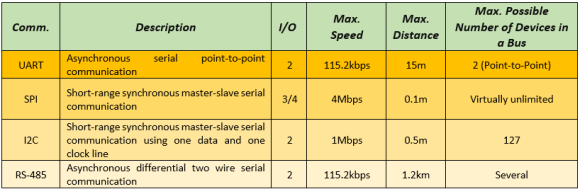N76E003 has two hardware UARTs, one SPI and one I2C peripheral. The UART interfaces can be further expanded to implement RS-485 and RS-422 communication protocols. With ordinary GPIOs and optionally with hardware timers we can implement software-based UART, SPI and I2C too. However, software methods are slow and resource-hungry. Software-based approach is, on the other hand, needed when we have to interface devices that don’t use any of these communication topologies. For example, the one-wire protocol used by DHT series relative humidity and temperature sensors needs to be implemented by using an ordinary GPIO pin. Same goes for typical text and graphical LCDs.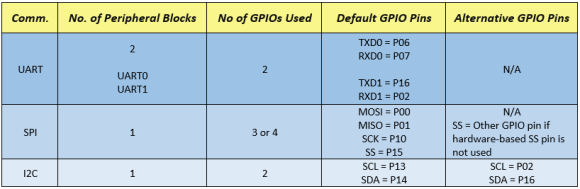Note that there is a conflict between UART1 and alternative I2C pins. There are also similar conflicts with other hardware peripherals like PWMs, ADCs, etc since N76E003 packs lots of stuff in such a small form-factor. Every single GPIO pin is precious. Try to keep things with default GPIO pins in order to reduce conflicts with other hardware peripherals, coding and to maximize effective use of GPIOs. Likewise bear in mind that timers have also such conflicts as they are used to implement both hardware-based delays and the UART peripherals. You have to know for sure what you are doing, what do you want and with which stuffs you wish to accomplish your task.

# Serial Communication – UART

To date serial communication (UART) is perhaps the simplest and widely used form of communication in use. UART block is only block available in most microcontrollers that can be easily interfaced with a computer or a phone. Most communication modules like Bluetooth, Wi-Fi, GSM modems, GPS modules, etc are interfaced using UART. It has incredible range and is also the backbone of other communication methods like RS-485, RS-422, etc.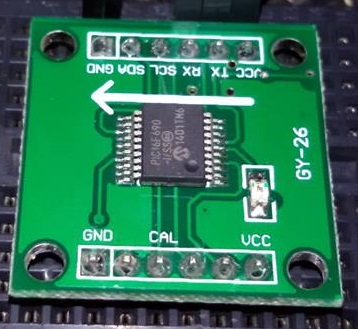https://learn.mikroe.com/uart-serial-communication

## Code

### HMC1022.h

`#define Get_Angular_Measurement                      0x31`
`#define Start_Calibration                            0xC0 `
`#define End_Calibration                              0xC1                     `
`#define Set_Magnetic_Declination_High_Byte           0x03  `
`#define Set_Magnetic_Declination_Low_Byte            0x04`

`#define no_of_data_bytes_returned                    0x08`

`#define calibration_LED                              P15`

`void read_heading(void);`
`void calibrate_compass(void); `
`void factory_reset(void);`
`void set_declination_angle(unsigned long angle);`

### HMC1022.c

`#include "N76E003.h"`
`#include "SFR_Macro.h"`
`#include "Function_define.h"`
`#include "Common.h"`
`#include "Delay.h"`
`#include "soft_delay.h"`
`#include "HMC1022.h"`

`unsigned char done = 0;`
`unsigned char data_bytes[no_of_data_bytes_returned] = {0x00, 0x00, 0x00, 0x00, 0x00, 0x00};`

`void read_heading(void)`
`{                        `
`     unsigned char s = 0; `
`     unsigned long CRC = 0;`

`     Send_Data_To_UART1(Get_Angular_Measurement); `

`     for(s = 0; s < no_of_data_bytes_returned; s++)`
`     {               `
`        data_bytes[s] = Receive_Data_From_UART1();`
`        if(s < (no_of_data_bytes_returned - 1))  `
`        {`
`            CRC += data_bytes[s];`
`        }`
`     }                                                      `

`     CRC = (CRC & 0xFF);`

`     if(CRC == data_bytes)`
`     {                                    `
`       done = 1;`
`     }    `
`}`

`void calibrate_compass(void)`
`{`
` unsigned char s = 0x00;    `

` Send_Data_To_UART1(Start_Calibration);`

` for(s = 0; s < 60; s++)`
` {`
`     calibration_LED = 1;       `
`     delay_ms(100);`
`     calibration_LED = 0;`
`     delay_ms(900);`
` } `

` for(s = 0; s < 60; s++)   `
` {`
`     calibration_LED = 1;   `
`     delay_ms(400);            `
`     calibration_LED = 0;`
`     delay_ms(600);`
` }             `

` Send_Data_To_UART1(End_Calibration);`
`}`

`void factory_reset(void)`
`{`
` Send_Data_To_UART1(0xA0);`
` Send_Data_To_UART1(0xAA);`
` Send_Data_To_UART1(0xA5); `
` Send_Data_To_UART1(0xC5);`
`}     `

`void set_declination_angle(unsigned long angle)`
`{`
` unsigned long hb = 0;`
` unsigned char lb = 0;`

` lb = (angle & 0x00FF);   `

` hb = (angle & 0xFF00);`
` hb >>= 8; `

` Send_Data_To_UART1(Set_Magnetic_Declination_High_Byte);    `
` Send_Data_To_UART1(hb);`

` Send_Data_To_UART1(Set_Magnetic_Declination_Low_Byte);`
` Send_Data_To_UART1(lb);`
`}`

### main.c

`#include "N76E003.h"`
`#include "SFR_Macro.h"`
`#include "Function_define.h"`
`#include "Common.h"`
`#include "Delay.h"`
`#include "soft_delay.h"`
`#include "LCD_2_Wire.h"`
`#include "HMC1022.h"`

`const unsigned char symbol =`
`{`
`   0x00, 0x06, 0x09, 0x09, 0x06, 0x00, 0x00, 0x00`
`};`

`extern unsigned char done;`
`extern unsigned char data_bytes[no_of_data_bytes_returned];`

`void setup(void);`
`void lcd_symbol(void);`

`void main(void)`
`{`
`  setup();`

`  while(1)`
`  {`
`    read_heading();`

`    if(done)`
`     {`
`       LCD_goto(6, 1);`
`       LCD_putchar(data_bytes);`

`       LCD_goto(7, 1);`
`       LCD_putchar(data_bytes);`

`       LCD_goto(8, 1);`
`       LCD_putchar(data_bytes);`

`       LCD_goto(9, 1);`
`       LCD_putchar('.');`
`       LCD_goto(10, 1);`
`       LCD_putchar(data_bytes);`

`       done = 0;`
`     }`

`     P15 = ~P15;`
`     delay_ms(200);`
`  };`
`}`

`void setup(void)`
`{`
`  P15_PushPull_Mode;`

`  LCD_init();`
`  LCD_clear_home();`
`  lcd_symbol();`
`  LCD_goto(3, 0);`
`  LCD_putstr("Heading  N");`
`  LCD_goto(11, 0);`
`  LCD_send(0, DAT);`

`  InitialUART1_Timer3(9600);`
`}`

`void lcd_symbol(void) `
`{`
`  unsigned char s = 0; `

`  LCD_send(0x40, CMD);`

`  for(s = 0; s < 8; s++)`
`  {`
`   LCD_send(symbol[s], DAT);`
`  }`

`  LCD_send(0x80, CMD);`
`}`

## Schematic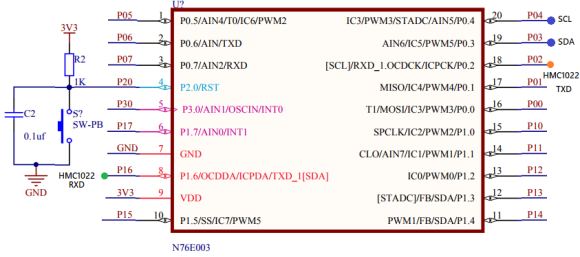## Explanation

The following are the commands that HMC1022 acknowledges when sent via UART:HMC1022 gives heading data when it is requested. The highlighted command is that command.

Once requested it sends out a string of characters that carry heading information. The followings are the response package and its details: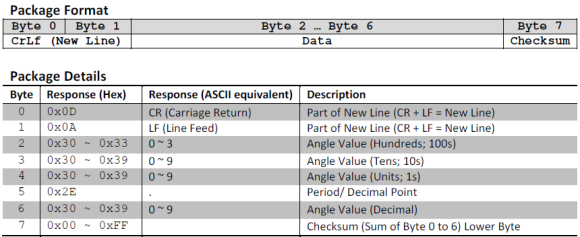From the above info, it is clear that HMC1022 will return 8 bytes when requested for heading. Out of these we need bytes 2, 3,4 and 6. The rest can be ignored for simplicity.

Data from HM1022 is received by the following function:

`void read_heading(void)`
`{                        `
`     unsigned char s = 0; `
`     unsigned long CRC = 0;`

`     Send_Data_To_UART1(Get_Angular_Measurement); `

`     for(s = 0; s < no_of_data_bytes_returned; s++)`
`     {               `
`        data_bytes[s] = Receive_Data_From_UART1();`
`        if(s < (no_of_data_bytes_returned - 1))  `
`        {`
`            CRC += data_bytes[s];`
`        }`
`     }                                                      `

`     CRC = (CRC & 0xFF);`

`     if(CRC == data_bytes)`
`     {                                    `
`       done = 1;`
`     }    `
`}`

Note that the function Send_Data_To_UART1 and Receive_Data_From_UART1 are BSP functions. Likewise, InitialUART1_Timer3(9600) function is also a BSP function that initiates UART1 with Timer 3. As with ADC, BSP functions for UART are enough for basic setup. Try to use Timer 3 as it remains mostly free. If you need more control over the UART, you can manually configure the registers.

## Demo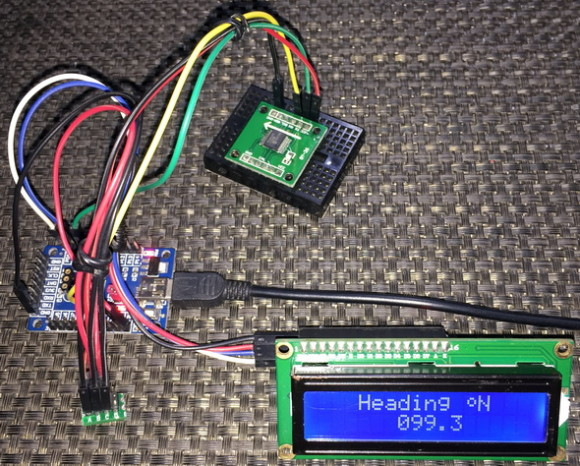# UART Interrupt

UART, as we saw in the last example, can be used in polling mode but it is wise to use it in interrupt mode. This feature becomes especially important and therefore, a must-have requirement when it come to that fact that the host micro may not always know when to receive data. Unlike SPI/I2C, UART doesn’t always follow known data transactions. Take the example of a GSM modem. We and so does our tiny host N76E003 micro don’t know for sure when a message would arrive. Thus, when an interrupt due to reception is triggered, it is known to mark the beginning of data reception process. The host micro can relax idle until data is received fully.

Here, we will see how to use UART interrupt to read a LV-Max EZ0 SONAR module.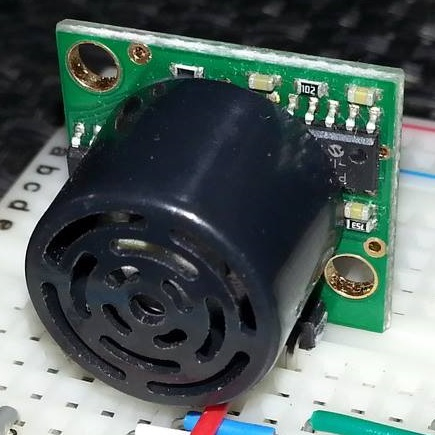## Code

`#include "N76E003.h"`
`#include "SFR_Macro.h"`
`#include "Function_define.h"`
`#include "Common.h"`
`#include "soft_delay.h"`
`#include "LCD_2_Wire.h"`

`unsigned char received = 0;`
`unsigned char count = 0;`
`unsigned char buffer = {0x00, 0x00, 0x00, 0x00, 0x00};`

`void setup(void);`

`void UART0_ISR(void)    `
`interrupt 4 `
`{`
`    if(RI == 1)`
`    {                                                               `
`        buffer[count] = SBUF;`
`        count++;`

`        if(count >= 4)`
`        {`
`            clr_ES;`
`            received = 1;`
`        }`

`        clr_RI;`
`    }`

`    P15 = ~P15;`
`}`

`void main(void)`
`{`
`    unsigned char i = 0;`

`    setup();`

`    while(1)`
`    {`
`        Send_Data_To_UART0('S');`
`        delay_ms(40);`
`        set_ES;     `

`        if(received)`
`        {`

`            for(i = 1; i < 4; i++)`
`            {`
`              LCD_goto((12 + i), 1);`
`              LCD_putchar(buffer[i]);`
`            }`

`            count = 0;`
`            received  = 0;`
`            set_ES;`
`        }`

`        delay_ms(40);`
`    }`
`}`

`void setup(void)`
`{`
`    P15_PushPull_Mode;`

`    LCD_init();`
`    LCD_clear_home();`

`    LCD_goto(1, 0);`
`    LCD_putstr("UART Interrupt");`
`    LCD_goto(0, 1);`
`    LCD_putstr("Range/Inch:");`

`    InitialUART0_Timer3(9600);`
`    set_EA;`
`}`

## Schematic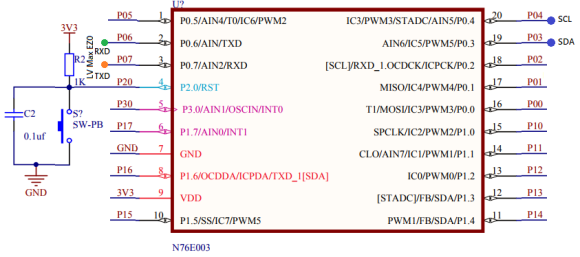## Explanation

This time UART0 is used and is setup using BSP built-in function. The only exception is the interrupt part. The global interrupt is enabled but not the UART interrupt.

`InitialUART0_Timer3(9600);`
`set_EA;`

The UART interrupt is only enabled after commanding the SONAR sensor:

`Send_Data_To_UART0('S');`
`delay_ms(40);`
`set_ES;  `

This is done to avoid unnecessary reads.

The sensor is read inside the interrupt routine. The sensor outputs data as Rxxx<CR>. So, there are 5 bytes to read. The “R” in the readout works as a preamble or sync byte. The “xxx” part contains range info in inches. Finally, <CR> is a carriage return character. Therefore, every time a character is received an interrupt is triggered and the character is saved in an array. When all 5 bytes have been received, the display is updated. On exiting the interrupt, the interrupt flag is cleared. P15 is also toggled to visually indicate that an UART interrupt has been triggered.

`void UART0_ISR(void)    `
`interrupt 4 `
`{`
`  if(RI == 1)`
`  {                                                               `
`     buffer[count] = SBUF;`
`     count++;`

`     if(count >= 4)`
`     {`
`       clr_ES;`
`       received = 1;`
`     }`

`     clr_RI;`
`  }`

`  P15 = ~P15;`
`}`

## Demo# Inter-Integrated Circuit (I2C) – Interfacing DS1307 I2C RTC

Developed by Philips nearly three decades ago, I2C communication (Inter-Integrated Circuit) is widely used in a number of devices and is comparatively easier than SPI. For I2C communication only two wires – serial data (SDA) and serial clock (SCK) are needed and these two wires form a bus in which we can connect up to 127 devices.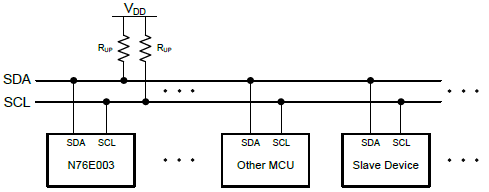Everything you need to know about I2C can be found in these pages:

Apart from these N76E003’s datasheet explains I2C communication in high detail.

## Code

### I2C.h

`#define regular_I2C_pins              0`
`#define alternate_I2C_pins            1`

`#define regular_I2C_GPIOs()           do{P13_OpenDrain_Mode; P14_OpenDrain_Mode; clr_I2CPX;}while(0)`

`#define alternative_I2C_GPIOs()       do{P02_OpenDrain_Mode; P16_OpenDrain_Mode; set_I2CPX;}while(0)`

`#define I2C_GPIO_Init(mode)           do{if(mode != 0){alternative_I2C_GPIOs();}else{regular_I2C_GPIOs();}}while(0)`

`#define I2C_CLOCK                     0x27 //Fclk = Fsys / (4*(prescalar + 1))`

`#define I2C_ACK                       0`
`#define I2C_NACK                      1`

`#define timeout_count                 1000`

`void I2C_init(void);`
`void I2C_start(void);`
`void I2C_stop(void);`
`unsigned char I2C_read(unsigned char ack_mode);`
`void I2C_write(unsigned char value);`

### I2C.c

`#include "N76E003.h"`
`#include "SFR_Macro.h"`
`#include "Function_define.h"`
`#include "Common.h"`
`#include "Delay.h"`
`#include "I2C.h"`

`void I2C_init(void)`
`{`
`    I2C_GPIO_Init(regular_I2C_pins);`
`    I2CLK = I2C_CLOCK;`
`    set_I2CEN;`
`}`

`void I2C_start(void)`
`{`
`    signed int t = timeout_count;`

`    set_STA;                               `
`    clr_SI;`
`    while((SI == 0) && (t > 0))`
`    {`
`       t--;`
`    };    `
`}`

`void I2C_stop(void)`
`{`
`    signed int t = timeout_count;`

`    clr_SI;`
`    set_STO;`
`    while((STO == 1) && (t > 0))`
`    {`
`        t--;`
`    };    `
`}`

`unsigned char I2C_read(unsigned char ack_mode)`
`{`
`    signed int t = timeout_count;`
`    unsigned char value = 0x00;`

`    set_AA;                            `
`    clr_SI;`
`    while((SI == 0) && (t > 0))`
`    {`
`        t--;`
`    };      `

`    value = I2DAT;`

`    if(ack_mode == I2C_NACK)`
`    {`
`        t = timeout_count;`
`        clr_AA;  `
`        clr_SI;`
`        while((SI == 0) && (t > 0))`
`        {`
`            t--;`
`        };          `
`    }`

`    return value;`
`}`

`void I2C_write(unsigned char value)`
`{`
`    signed int t = timeout_count;`

`    I2DAT = value;`
`    clr_STA;          `
`    clr_SI;`
`    while((SI == 0) && (t > 0))`
`    {`
`        t--;`
`    }; `
`}`

### DS1307.h

`#define I2C_W                            0`
`#define I2C_R                            1`

`#define sec_reg                          0x00`
`#define min_reg                          0x01`
`#define hr_reg                           0x02`
`#define day_reg                          0x03`
`#define date_reg                         0x04`
`#define month_reg                        0x05`
`#define year_reg                         0x06`
`#define control_reg                      0x07`

`#define DS1307_addr                      0xD0`
`#define DS1307_WR                        (DS1307_addr + I2C_W)`
`#define DS1307_RD                        (DS1307_addr + I2C_R)`

`void DS1307_init(void);`
`unsigned char DS1307_read(unsigned char address);`
`void DS1307_write(unsigned char address, unsigned char value);`
`unsigned char bcd_to_decimal(unsigned char value);`
`unsigned char decimal_to_bcd(unsigned char value);`
`void get_time(void);`
`void set_time(void);`

### DS1307.c

`#include "N76E003.h"`
`#include "SFR_Macro.h"`
`#include "Function_define.h"`
`#include "Common.h"`
`#include "Delay.h"`
`#include "DS1307.h"`
`#include "I2C.h"`

`struct`
`{`
`   unsigned char s;`
`   unsigned char m;`
`   unsigned char h;`
`   unsigned char dy;`
`   unsigned char dt;`
`   unsigned char mt;`
`   unsigned char yr;`
`}time;`

`void DS1307_init(void)`
`{`
`    I2C_init();`
`  DS1307_write(control_reg, 0x00);`
`}`

`unsigned char DS1307_read(unsigned char address)`
`{`
`    unsigned char value = 0x00;`

`    I2C_start();`
`    I2C_write(DS1307_WR);`
`    I2C_write(address);`

`    I2C_start();`
`    I2C_write(DS1307_RD);`
`    value = I2C_read(I2C_NACK);`
`    I2C_stop();`

`    return value;`
`}`

`void DS1307_write(unsigned char address, unsigned char value)`
`{`
`    I2C_start();`
`    I2C_write(DS1307_WR);`
`    I2C_write(address);`
`    I2C_write(value);`
`    I2C_stop();`
`}`

`unsigned char bcd_to_decimal(unsigned char value)`
`{`
`    return ((value & 0x0F) + (((value & 0xF0) >> 0x04) * 0x0A));`
`}`

`unsigned char decimal_to_bcd(unsigned char value)`
`{`
`    return (((value / 0x0A) << 0x04) & 0xF0) | ((value % 0x0A) & 0x0F);`
`}`

`void get_time(void)`
`{`
`    time.s = DS1307_read(sec_reg);`
`    time.s = bcd_to_decimal(time.s);`

`    time.m = DS1307_read(min_reg);`
`    time.m = bcd_to_decimal(time.m);`

`    time.h = DS1307_read(hr_reg);`
`    time.h = bcd_to_decimal(time.h);`

`    time.dy = DS1307_read(day_reg);`
`    time.dy = bcd_to_decimal(time.dy);`

`    time.dt = DS1307_read(date_reg);`
`    time.dt = bcd_to_decimal(time.dt);`

`    time.mt = DS1307_read(month_reg);`
`    time.mt = bcd_to_decimal(time.mt);`

`    time.yr = DS1307_read(year_reg);`
`    time.yr = bcd_to_decimal(time.yr);`
`}`

`void set_time(void)`
`{`
`    time.s = decimal_to_bcd(time.s);`
`    DS1307_write(sec_reg, time.s);`

`    time.m = decimal_to_bcd(time.m);`
`    DS1307_write(min_reg, time.m);`

`    time.h = decimal_to_bcd(time.h);`
`    DS1307_write(hr_reg, time.h);`

`    time.dy = decimal_to_bcd(time.dy);`
`    DS1307_write(day_reg, time.dy);`

`    time.dt = decimal_to_bcd(time.dt);`
`    DS1307_write(date_reg, time.dt);`

`    time.mt = decimal_to_bcd(time.mt);`
`    DS1307_write(month_reg, time.mt);`

`    time.yr = decimal_to_bcd(time.yr);`
`    DS1307_write(year_reg, time.yr);`
`}`

### main.c

`#include "N76E003.h"`
`#include "SFR_Macro.h"`
`#include "Function_define.h"`
`#include "Common.h"`
`#include "Delay.h"`
`#include "soft_delay.h"`
`#include "LCD_3_Wire.h"`
`#include "I2C.h"`
`#include "DS1307.h"`

`extern struct`
`{`
`   unsigned char s;`
`   unsigned char m;`
`   unsigned char h;`
`   unsigned char dy;`
`   unsigned char dt;`
`   unsigned char mt;`
`   unsigned char yr;`
`}time;`

`void show_value(unsigned char x_pos, unsigned char y_pos, unsigned char value);`
`void display_time(void);`

`void main(void)`
`{`
`    time.s = 30;`
`    time.m = 58;`
`    time.h = 23;`

`    P15_PushPull_Mode;`

`    LCD_init();`
`    LCD_clear_home();`

`    LCD_goto(0, 0);`

`    LCD_putstr("N76E003 I2C RTCC");`

`    DS1307_init();`
`    set_time();`

`    while(1)`
`    {`
`        get_time();`
`        display_time();`
`    };`
`}`

`void show_value(unsigned char x_pos, unsigned char y_pos, unsigned char value)`
`{`
`    unsigned char chr = 0;`

`    chr = ((value / 10) + 0x30);`
`    LCD_goto(x_pos, y_pos);`
`    LCD_putchar(chr);`

`    chr = ((value % 10) + 0x30);`
`    LCD_goto((x_pos + 1), y_pos);`
`    LCD_putchar(chr);`
`}`

`void display_time(void)`
`{`
`    P15 ^= 1;`
`    LCD_goto(6, 1);`
`    LCD_putchar(' ');`
`    LCD_goto(9, 1);`
`    LCD_putchar(' ');`
`    delay_ms(400);`

`    show_value(4, 1, time.h);`
`    show_value(7, 1, time.m);`
`    show_value(10, 1, time.s);`

`    LCD_goto(6, 1);`
`    LCD_putchar(':');`
`    LCD_goto(9, 1);`
`    LCD_putchar(':');`
`    delay_ms(400);`
`}`

## Schematic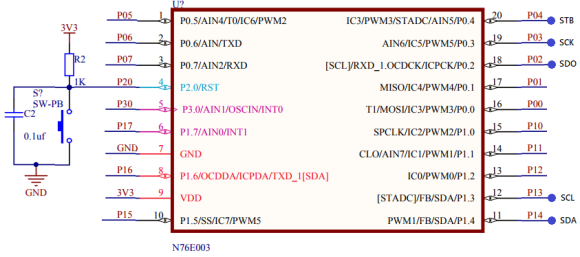## Explanation

I have coded two files for I2C easy and quick I2C implementation. These are I2C header and source file. These files describe I2C hardware functionality as well as provide higher level functions under the hood of which I2C hardware is manipulated. I have only coded these files for master mode only since barely we would ever need N76E003 slaves. I also didn’t implement interrupt-based I2C functionalities as this, in my experience, is also not needed in most of the cases.

`void I2C_init(void);`
`void I2C_start(void);`
`void I2C_stop(void);`
`unsigned char I2C_read(unsigned char ack_mode);`
`void I2C_write(unsigned char value);`

Using hardware I2C requires us to manipulate the following registers apart from clock and GPIO settings.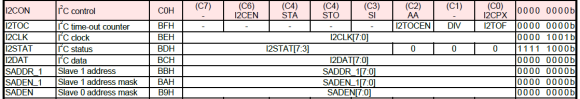I2C GPIOs must be set as open-drain GPIOs as per I2C standard. I2C clock must be set according to the devices connected in the bus. Typically, I2C clock speed ranges from 20 – 400kHz. This clock speed is dependent on system clock speed Fsys. Therefore, before setting I2C clock speed you have to set/know Fsys. I2C clock is decided by the value of I2CLK register. It is basically a prescalar value in master mode.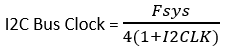So, if Fsys = 16MHz and I2CLK = 39 (0x27), the I2C bus clock rate is 100kHz.

Note that only in master I2C clock can be setup. In slave mode, the slave(s) synchronizes automatically with bus clock.

I2CON register is they key register for using I2C. It contains all the flags and condition-makers.

For instance, consider the I2C start condition. In order to make a start condition, we have to set the STA bit and clear I2C interrupt flag, SI. Since I used polling mode, SI is polled until it is cleared. This ensures that a start condition has been successfully made. I have also added a software-based timeout feature should there be an issue with the I2C bus. In N76E003, there is a hardware-based approach for timeout too. Note that I didn’t care about the I2C status register states as again this is rarely needed. If you want more grip on the I2C you can follow the BSP examples.

`void I2C_start(void)`
`{`
`    signed int t = timeout_count;`

`    set_STA;                               `
`    clr_SI;`
`    while((SI == 0) && (t > 0))`
`    {`
`       t--;`
`    };    `
`}`

Finally, the last register that we will need is the I2C data register (I2DAT). It is through it we send and receive data from I2C bus.

The demo I have shown here is that of a DS1307 I2C-based real time clock. Perhaps this is the simplest device for learning about and understanding I2C.

## Demo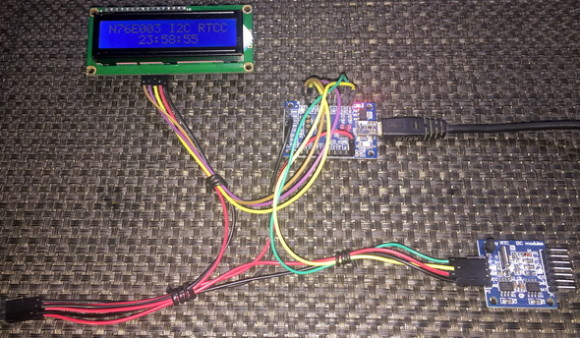# Serial Peripheral Interface (SPI) – Interfacing MAX7219 and MAX6675

SPI just like I2C is another highly popular form of onboard serial communication. Compared to I2C it is fast and robust. However, it requires more GPIO pins than other communication forms. These make it ideal communication interface for TFT displays, OLED displays, flash memories, DACs, etc.

SPI is best realized as a shift register that shifts data in and out with clock pulses. In a SPI bus, there is always one master device which generates clock and selects slave(s). Master sends commands to slave(s). Slave(s) responds to commands sent by the master. The number of slaves in a SPI bus is virtually unlimited. Except the chip selection pin, all SPI devices in a bus can share the same clock and data pins.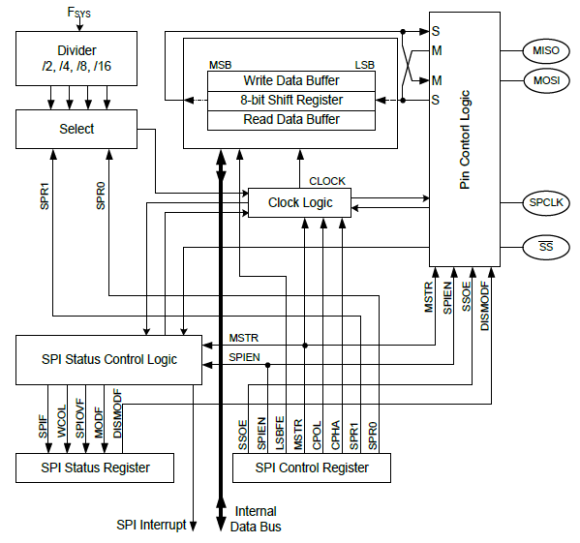In general, if you wish to know more about SPI bus here are some cool links:

## Code

### MAX72xx.h

`#define MAX72xx_SPI_GPIO_init()                             do{P00_PushPull_Mode; P10_PushPull_Mode; P11_PushPull_Mode;}while(0)`

`#define MAX72xx_CS_OUT_HIGH()                               set_P11`
`#define MAX72xx_CS_OUT_LOW()                                clr_P11`

`#define NOP                                                 0x00`
`#define DIG0                                                0x01`
`#define DIG1                                                0x02`
`#define DIG2                                                0x03`
`#define DIG3                                                0x04`
`#define DIG4                                                0x05`
`#define DIG5                                                0x06`
`#define DIG6                                                0x07`
`#define DIG7                                                0x08`
`#define decode_mode_reg                                     0x09`
`#define intensity_reg                                       0x0A`
`#define scan_limit_reg                                      0x0B`
`#define shutdown_reg                                        0x0C`
`#define display_test_reg                                    0x0F`

`#define shutdown_cmd                                        0x00`
`#define run_cmd                                             0x01`

`#define no_test_cmd                                         0x00`
`#define test_cmd                                            0x01`

`#define digit_0_only                                        0x00`
`#define digit_0_to_1                                        0x01`
`#define digit_0_to_2                                        0x02`
`#define digit_0_to_3                                        0x03`
`#define digit_0_to_4                                        0x04`
`#define digit_0_to_5                                        0x05`
`#define digit_0_to_6                                        0x06`
`#define digit_0_to_7                                        0x07`

`#define No_decode_for_all                                   0x00`
`#define Code_B_decode_digit_0                               0x01`
`#define Code_B_decode_digit_0_to_3                         0x0F    `
`#define Code_B_decode_for_all                               0xFF`

`void MAX72xx_SPI_HW_Init(unsigned char clk_value);`
`void MAX72xx_init(void);`
`void MAX72xx_write(unsigned char address, unsigned char value);`

### MAX72xx.c

`#include "N76E003_IAR.h"`
`#include "SFR_Macro.h"`
`#include "Function_define.h"`
`#include "Common.h"`
`#include "Delay.h"`
`#include "MAX72xx.h"`

`void MAX72xx_SPI_HW_Init(unsigned char clk_value)`
`{`
`  switch(clk_value)`
`  {`
`    case 1:`
`    {`
`      clr_SPR1;`
`      set_SPR0;`
`      break;`
`    }`
`    case 2:`
`    {`
`      set_SPR1;`
`      clr_SPR0;`
`      break;`
`    }`
`    case 3:`
`    {`
`      set_SPR1;`
`      set_SPR0;`
`      break;`
`    }`
`    default:`
`    {`
`      clr_SPR1;`
`      clr_SPR0;`
`      break;`
`    }`
`  }   `

`  set_DISMODF;`
`  set_MSTR;`
`  clr_CPOL;`
`  clr_CPHA;`
`  set_SPIEN;`
`}`

`void MAX72xx_init(void)`
`{`
`  MAX72xx_SPI_GPIO_init();`
`  MAX72xx_SPI_HW_Init(0);`

`  MAX72xx_write(shutdown_reg, run_cmd);`
`  MAX72xx_write(decode_mode_reg, Code_B_decode_digit_0_to_3);`
`  MAX72xx_write(scan_limit_reg, digit_0_to_3);`
`  MAX72xx_write(intensity_reg, 0x19);`
`}`

`void MAX72xx_write(unsigned char address, unsigned char value)`
`{`
`  MAX72xx_CS_OUT_LOW(); `

`  SPDR = address;`
`  while(!(SPSR & SET_BIT7)); `
`  clr_SPIF;`

`  SPDR = value;`
`  while(!(SPSR & SET_BIT7)); `
`  clr_SPIF;`

`  MAX72xx_CS_OUT_HIGH();`
`}`

### MAX6675.h

`#define MAX6675_SPI_GPIO_init()     do{P01_Input_Mode; P10_PushPull_Mode; P12_PushPull_Mode;}while(0)`

`#define MAX6675_CS_OUT_HIGH()       set_P12`
`#define MAX6675_CS_OUT_LOW()        clr_P12`

`#define T_min                       0`
`#define T_max                       1024`

`#define count_max                   4096`

`#define no_of_pulses                16`

`#define deg_C                       0`
`#define deg_F                       1`
`#define tmp_K                       2`

`#define open_contact                0x04`
`#define close_contact               0x00`

`#define scalar_deg_C                0.25`
`#define scalar_deg_F_1              1.8`
`#define scalar_deg_F_2              32.0`
`#define scalar_tmp_K                273.0`

`#define no_of_samples               16`

`void MAX6675_SPI_HW_Init(unsigned char clk_value);`
`void MAX6675_init(void);`
`unsigned char MAX6675_get_ADC(unsigned int *ADC_data);`
`float MAX6675_get_T(unsigned int ADC_value, unsigned char T_unit);`

### MAX6675.c

`#include "N76E003_IAR.h"`
`#include "SFR_Macro.h"`
`#include "Function_define.h"`
`#include "Common.h"`
`#include "Delay.h"`
`#include "MAX6675.h"`

`void MAX6675_SPI_HW_Init(unsigned char clk_value)`
`{`
`  switch(clk_value)`
`  {`
`    case 1:`
`    {`
`      clr_SPR1;`
`      set_SPR0;`
`      break;`
`    }`
`    case 2:`
`    {`
`      set_SPR1;`
`      clr_SPR0;`
`      break;`
`    }`
`    case 3:`
`    {`
`      set_SPR1;`
`      set_SPR0;`
`      break;`
`    }`
`    default:`
`    {`
`      clr_SPR1;`
`      clr_SPR0;`
`      break;`
`    }`
`  }   `

`  set_DISMODF;`
`  set_MSTR;`
`  clr_CPOL;`
`  set_CPHA;`
`  set_SPIEN;`
`}`

`void MAX6675_init(void)`
`{`
`    MAX6675_SPI_GPIO_init();`
`    MAX6675_SPI_HW_Init(0);`
`}`

`unsigned char MAX6675_get_ADC(unsigned int *ADC_data)`
`{`
`    unsigned char lb = 0;`
`    unsigned char hb = 0;`
`    unsigned char samples = no_of_samples;`
`    unsigned int temp_data = 0;`
`    unsigned long avg_value = 0;`

`    while(samples > 0)`
`    {`
`         MAX6675_CS_OUT_LOW();`

`         SPDR = 0x00;`
`         while(!(SPSR & SET_BIT7)); `
`         hb = SPDR;`
`         clr_SPIF; `

`         SPDR = 0x00;`
`         while(!(SPSR & SET_BIT7));         `
`         lb = SPDR;`
`         clr_SPIF;   `

`         MAX6675_CS_OUT_HIGH();`

`         temp_data = hb;`
`         temp_data <<= 8;`
`         temp_data |= lb;`
`         temp_data &= 0x7FFF;`

`         avg_value += (unsigned long)temp_data;`

`         samples--;`
`         Timer0_Delay1ms(10);`
`    };`

`    temp_data = (avg_value >> 4);`

`    if((temp_data & 0x04) == close_contact)`
`    {`
`        *ADC_data = (temp_data >> 3);`
`        return close_contact;`
`    }`
`    else`
`    {`
`        *ADC_data = (count_max + 1);`
`        return open_contact;`
`    }`
`}`

`float MAX6675_get_T(unsigned int ADC_value, unsigned char T_unit)`
`{`
`    float tmp = 0.0;`

`    tmp = (((float)ADC_value) * scalar_deg_C);`

`    switch(T_unit)`
`    {`
`        case deg_F:`
`        {`
`             tmp *= scalar_deg_F_1;`
`             tmp += scalar_deg_F_2;`
`             break;`
`        }`
`        case tmp_K:`
`        {`
`            tmp += scalar_tmp_K;`
`            break;`
`        }`
`        default:`
`        {`
`            break;`
`        }`
`    }`

`    return tmp;`
`}`

### main.c

`#include "N76E003_IAR.h"`
`#include "Common.h"`
`#include "Delay.h"`
`#include "SFR_Macro.h"`
`#include "Function_define.h"`
`#include "soft_delay.h"`
`#include "MAX72xx.h"`
`#include "MAX6675.h"`

`void main(void)`
`{`
`  unsigned int ti = 0;`
`  unsigned int t = 0;`

`  P15_PushPull_Mode;`
`  MAX6675_init();`
`  MAX72xx_init(); `

`  while(1)`
`  {`
`      P15 = 1;`
`      clr_CPOL;`
`      set_CPHA;`
`      MAX6675_get_ADC(&ti);`
`      t = ((unsigned int)MAX6675_get_T(ti, tmp_K));`
`      delay_ms(100);`

`      P15 = 0;`
`      clr_CPOL;`
`      clr_CPHA;`
`      MAX72xx_write(DIG3, ((t / 1000) % 10));`
`      MAX72xx_write(DIG2, ((t / 100) % 10));`
`      MAX72xx_write(DIG1, ((t / 10) % 10));`
`      MAX72xx_write(DIG0, (t % 10));`
`      delay_ms(100);`
`  };`
`}`

## Schematic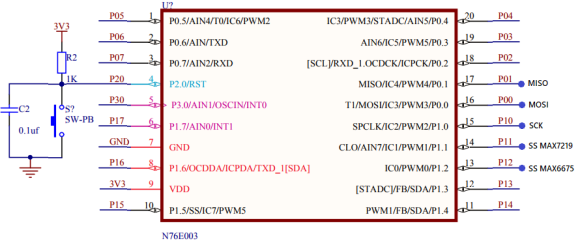## Explanation

With SPI we have to deal with two things – first, the initialization and second, the data transfer process. Configuring SPI needs a few things to be set up. These are:

• SPI communication bus speed or simply clock speed
• Clock polarity
• Clock phase
• Orientation of data, i.e. MSB first or LSB first
• Master/slave status
• Optionally hardware slave selection pin use

Most of these can be done by manipulating the following registers:SPCR configures the hardware SPI peripheral of N76E003 while SPDR is used for transferring data.

To aid in all these I have coded the following functions. Though I didn’t use these code snippets in the actual demo code for demonstration purposes, these will most certainly work and reduce coding effort.

`void SPI_init(unsigned char clk_speed, unsigned char mode)`
`{`
`  set_SPI_clock_rate(clk_speed);`
`  set_SPI_mode(mode);`
`  set_DISMODF;`
`  set_MSTR;`
`  set_SPIEN;`
```}

```
`unsigned char SPI_transfer(unsigned char write_value)`
`{`
`  signed int t = timeout_count;`
`  register unsigned char read_value = 0x00;`

`  SPDR = write_value;`
`  while((!(SPSR & SET_BIT7)) && (t > 0))`
`  {`
`    t--;`
`  };        `
`  read_value = SPDR;`
`  clr_SPIF;   `

`  return read_value;`
`}`

SPI_init function sets up the SPI peripheral using two information – the bus clock speed and SPI data transfer mode. It initializes the SPI hardware as a SPI master device and without hardware salve selection pin. This configuration is mostly used in common interfacings. Only mode and clock speed are the variables. In the demo, it is visible that MAX7219 and MAX6675 work with different SPI modes. I also didn’t use the hardware slave selection because there are two devices and one slave pin. Certainly, both external devices are not driven at the same time although both share the same bus lines.

SPI_transfer reads and writes on the SPI bus. Here the master writes to the slave first, waits for successful transmission and reads from the slave later. It is best realized by the following figure:To demo SPI, MAX6675 and MAX7219 were used because one lacked read feature while the other lacked write feature. In the demo, MAX6675 is read to measure the temperature sensed by the K-type thermocouple attached with it and display the measured temperature in Kelvin on MAX7219-based seven segment display arrays.

## Demo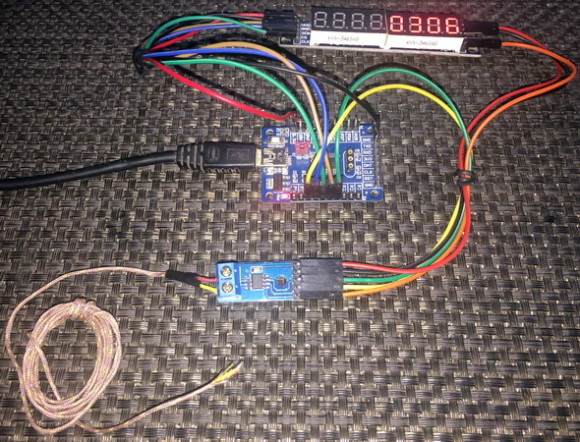# One Wire (OW) Communication – Interfacing DHT11

One protocol is not a standard protocol like I2C or SPI. It is just a mere method of communicating with a device that uses just one wire. There are a few devices that uses such principle for communication. DHT series sensors, DS18B20s, digital serial number chips, etc. are a few to mention. As I stated before, since one wire communication doesn’t belong to a dedicated format of communication, there is no dedicated hardware for it. With just a bidirectional GPIO pin we can implement one wire communication. Optionally we can also use a timer for measuring response pulse widths since the method of sending ones and zeroes over one wire requires the measurement of pulse durations. This way of encoding ones and zeros as a function of pulse width is called time-slotting.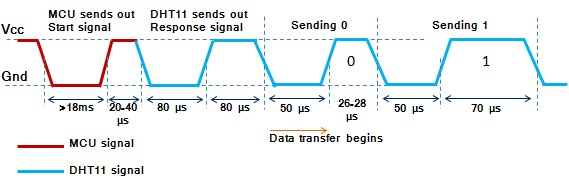Shown above is the timing diagram for DHT11’s time slots. Check the length of pulse high time for one and zero. This is the technique applied in time-slotting mechanism. We have to time pulse lengths in order to identify the logic states.

## Code

### DHT11.h

`#define HIGH                    1`
`#define LOW                     0`

`#define DHT11_pin_init()        P05_Quasi_Mode      `

`#define DHT11_pin_HIGH()        set_P05`
`#define DHT11_pin_LOW()         clr_P05`

`#define DHT11_pin_IN()          P05             `

`void DHT11_init(void);`
`unsigned char get_byte(void);`
`unsigned char get_data(void);`

### DHT11.c

`#include "N76E003.h"`
`#include "SFR_Macro.h"`
`#include "Function_define.h"`
`#include "Common.h"`
`#include "Delay.h"`
`#include "soft_delay.h"`
`#include "DHT11.h"`

`extern unsigned char values;`

`void DHT11_init(void)`
`{`
`   DHT11_pin_init();`
`   delay_ms(1000);`
`}`

`unsigned char get_byte(void)`
`{`
`   unsigned char s = 0;`
`   unsigned char value = 0;`

`   for(s = 0; s < 8; s++)`
`   {`
`      value <<= 1;`
`      while(DHT11_pin_IN() == LOW);`
`      delay_us(30);`

`      if(DHT11_pin_IN() == HIGH)`
`      {`
`          value |= 1;`
`      }`

`      while(DHT11_pin_IN() == HIGH);`
`   }`
`   return value;`
`}`

`unsigned char get_data(void)`
`{`
`   short chk = 0;`
`   unsigned char s = 0;`
`   unsigned char check_sum = 0;`

`   DHT11_pin_HIGH();`
`   DHT11_pin_LOW();`
`   delay_ms(18);`
`   DHT11_pin_HIGH();`
`   delay_us(26);`

`   chk = DHT11_pin_IN();`

`   if(chk)`
`   {`
`      return 1;`
`   }`
`   delay_us(80);`

`   chk = DHT11_pin_IN();`

`   if(!chk)`
`   {`
`      return 2;`
`   }`
`   delay_us(80);`

`   for(s = 0; s <= 4; s += 1)`
`   {`
`       values[s] = get_byte();`
`   }`

`   DHT11_pin_HIGH();`

`   for(s = 0; s < 4; s += 1)`
`   {`
`       check_sum += values[s];`
`   }`

`   if(check_sum != values)`
`   {`
`      return 3;`
`   }`
`   else`
`   {`
`      return 0;`
`   }`
`}`

### main.c

`#include "N76E003.h"`
`#include "SFR_Macro.h"`
`#include "Function_define.h"`
`#include "Common.h"`
`#include "Delay.h"`
`#include "soft_delay.h"`
`#include "LCD_2_Wire.h"`
`#include "DHT11.h"`

`unsigned char values;`

`const unsigned char symbol =`
`{`
`   0x00, 0x06, 0x09, 0x09, 0x06, 0x00, 0x00, 0x00`
`};`

`void setup(void);`
`void lcd_symbol(void);`
`void lcd_print(unsigned char x_pos, unsigned char y_pos, unsigned char value);`

`void main(void)`
`{`
`  unsigned char state = 0;`

`  setup();`

`  while(1)`
`  {`
`    state = get_data();`

`    switch(state)`
`    {`
`            case 1:`
`            {`
`            }`
`            case 2:`
`            {`
`                 LCD_clear_home();`
`                 LCD_putstr("No Sensor Found!");`
`                 break;`
`            }`
`            case 3:`
`            {`
`                 LCD_clear_home();`
`                 LCD_putstr("Checksum Error!");`
`                 break;`
`            }`
`            default:`
`            {`
`                 LCD_goto(0, 0);`
`                 LCD_putstr("R.H/ %:       ");`

`                 lcd_print(14, 0, values);`

`                 LCD_goto(0, 1);`
`                 LCD_putstr("Tmp/");`
`                 LCD_goto(4, 1);`
`                 LCD_send(0, DAT);`
`                 LCD_goto(5, 1);`
`                 LCD_putstr("C:");`

`                 if((values & 0x80) == 1)`
`                 {`
`                     LCD_goto(13, 1);`
`                     LCD_putstr("-");`
`                 }`
`                 else`
`                 {`
`                     LCD_goto(13, 1);`
`                     LCD_putstr(" ");`
`                 }`

`                 lcd_print(14, 1, values);`
`                 break;`
`            }`
`    }`

`    delay_ms(1000);`
`  };`
`}`

`void setup(void)`
`{`
`  DHT11_init();`

`  LCD_init(); `
`  LCD_clear_home();`
`  lcd_symbol();`
`}`

`void lcd_symbol(void) `
`{`
`  unsigned char s = 0; `

`  LCD_send(0x40, CMD);`

`  for(s = 0; s < 8; s++)`
`  {`
`   LCD_send(symbol[s], DAT);`
`  }`

`  LCD_send(0x80, CMD);`
`}`

`void lcd_print(unsigned char x_pos, unsigned char y_pos, unsigned char value)`
`{`
` unsigned char chr = 0x00;`

` chr = ((value / 10) + 0x30);`
` LCD_goto(x_pos, y_pos);`
` LCD_putchar(chr);`

` chr = ((value % 10) + 0x30);`
` LCD_goto((x_pos + 1), y_pos);`
` LCD_putchar(chr);`
`}`

## Schematic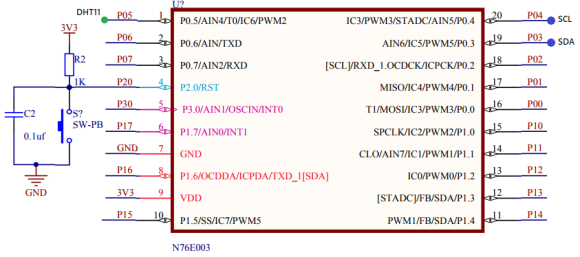## Explanation

As stated earlier, OW basically needs two things – first, the control of a single GPIO pin and second, the measurement of time. I would like to highlight the most important part of the whole code here:

`unsigned char get_byte(void)`
`{`
`   unsigned char s = 0;`
`   unsigned char value = 0;`

`   for(s = 0; s < 8; s++)`
`   {`
`      value <<= 1;`
`      while(DHT11_pin_IN() == LOW);`
`      delay_us(30);`

`      if(DHT11_pin_IN() == HIGH)`
`      {`
`          value |= 1;`
`      }`

`      while(DHT11_pin_IN() == HIGH);`
`   }`
`   return value;`
`}`

From DHT11’s timing diagram, we know that a zero is define by a pulse of 26 – 28μs and a logic one is defined by a pulse of 70μs. It is here in the get_byte function we check the pulse coming from DHT11. The DHT11’s pin is polled as an input and after 30μs if the result of this polling is high then it is considered as a logic one or else it is considered otherwise. Once triggered, DHT11 will provide a 5-byte output. All of the bits in these 5 bytes are checked this way. Though it is not the best method to do so, it is easy and simple.

## Demo# One Wire (OW) Communication – Interfacing DS18B20

One wire communication, as stated previously, doesn’t have a defined protocol. Except for the time-slotting mechanism part, there is nothing in common across the devices using this form of communication. We have seen how to interface a DHT11 relative humidity and temperature sensor previously. In this segment, we will see how to interface a DS18B20 one-wire digital temperature sensor with N76E003. DS18B20 follows and uses a completely different set of rules for communication.

## Code

### one_wire.h

`#define DS18B20_GPIO_init()             P06_OpenDrain_Mode`

`#define DS18B20_IN()                    P06`

`#define DS18B20_OUT_LOW()               clr_P06`
`#define DS18B20_OUT_HIGH()              set_P06`

`#define TRUE                            1`
`#define FALSE                           0`

`unsigned char onewire_reset(void);`
`void onewire_write_bit(unsigned char bit_value);`
`unsigned char onewire_read_bit(void);`
`void onewire_write(unsigned char value);   `
`unsigned char onewire_read(void);`

### one_wire.c

`#include "N76E003.h"`
`#include "SFR_Macro.h"`
`#include "Function_define.h"`
`#include "Common.h"`
`#include "Delay.h"`
`#include "soft_delay.h"`
`#include "one_wire.h"`
`unsigned char onewire_reset(void) `
`{                                        `
`     unsigned char res = FALSE;`
`     DS18B20_GPIO_init();`
`     DS18B20_OUT_LOW();`
`     delay_us(480);       `
`     DS18B20_OUT_HIGH();`
`     delay_us(60);       `
`     res = DS18B20_IN();`
`     delay_us(480);      `
`     return res;`
`}`
`void onewire_write_bit(unsigned char bit_value)`
`{`
`    DS18B20_OUT_LOW();`
`    if(bit_value)`
`    {       `
`        delay_us(104);`
`        DS18B20_OUT_HIGH();  `
`    }             `
`}    `
`unsigned char onewire_read_bit(void)       `
`{    `
`    DS18B20_OUT_LOW(); `
`    DS18B20_OUT_HIGH(); `
`  delay_us(15);`
`    return(DS18B20_IN());   `
`}`
`void onewire_write(unsigned char value)`
`{                   `
`     unsigned char s = 0;`
`     while(s < 8)   `
`     {                             `
`          if((value & (1 << s)))`
`          {`
`              DS18B20_OUT_LOW();`
`                nop;`
`              DS18B20_OUT_HIGH(); `
`              delay_us(60);  `
`          }      `
`          else`
`          {`
`            DS18B20_OUT_LOW();           `
`              delay_us(60);          `
`              DS18B20_OUT_HIGH();  `
`              nop;`
`          }`
`          s++;`
`     }`
`}                                     `
`unsigned char onewire_read(void)`
`{`
`     unsigned char s = 0x00;`
`     unsigned char value = 0x00;`
`     while(s < 8)`
`     {`
`          DS18B20_OUT_LOW();`
`          nop;`
`          DS18B20_OUT_HIGH(); `
`          if(DS18B20_IN()) `
`          {                                     `
`              value |=  (1 << s);                         `
`          }       `
`          delay_us(60);`
`          s++;`
`     }    `
`     return value;`
`}`

### DS18B20.h

`#include "one_wire.h" `

`#define convert_T                       0x44`
`#define read_scratchpad                 0xBE           `
`#define write_scratchpad                0x4E`
`#define copy_scratchpad                 0x48  `
`#define recall_E2                       0xB8`
`#define read_power_supply               0xB4   `
`#define skip_ROM                        0xCC`

`#define resolution                      12`

`void DS18B20_init(void);`
`float DS18B20_get_temperature(void); `

### DS18B20.c

`#include "N76E003.h"`
`#include "SFR_Macro.h"`
`#include "Function_define.h"`
`#include "Common.h"`
`#include "Delay.h"`
`#include "soft_delay.h"`
`#include "DS18B20.h"`

`void DS18B20_init(void)                            `
`{                                      `
`    onewire_reset();`
`    delay_ms(100);`
`}             `

`float DS18B20_get_temperature(void)`
`{                                              `
`    unsigned char msb = 0x00;`
`    unsigned char lsb = 0x00;`
`    register float temp = 0.0; `

`    onewire_reset();    `
`    onewire_write(skip_ROM);       `
`    onewire_write(convert_T);`

`    switch(resolution)  `
`    {                                                 `
`        case 12:`
`        {                                           `
`            delay_ms(750);`
`            break;`
`        }               `
`        case 11:                                    `
`        {             `
`            delay_ms(375);`
`            break;`
`        }          `
`        case 10:                            `
`        {                                `
`            delay_ms(188);  `
`            break;`
`        }                                       `
`        case 9:                                  `
`        {                                               `
`            delay_ms(94);                `
`            break;                           `
`        }                       `
`    }                 `

`    onewire_reset();`

`    onewire_write(skip_ROM);                `
`    onewire_write(read_scratchpad);`

`    lsb = onewire_read();`
`    msb = onewire_read();`

`    temp = msb;                          `
`    temp *= 256.0;`
`    temp += lsb;`

`    switch(resolution)  `
`    {                                 `
`        case 12:           `
`        {                                               `
`            temp *= 0.0625;                `
`            break;                           `
`        }       `
`        case 11:`
`        {          `
`            temp *= 0.125;      `
`            break;`
`        }               `
`        case 10:`
`        {           `
`            temp *= 0.25;       `
`            break;`
`        } `
`        case 9:                                `
`        {                                `
`            temp *= 0.5;        `
`            break;     `
`        }                         `
`    }  `

`    delay_ms(40);       `

`    return (temp);      `
`}`

### main.c

`#include "N76E003.h"`
`#include "SFR_Macro.h"`
`#include "Function_define.h"`
`#include "Common.h"`
`#include "Delay.h"`
`#include "soft_delay.h"`
`#include "DS18B20.h"`
`#include "LCD_3_Wire.h"`

`const unsigned char symbol =`
`{`
`   0x00, 0x06, 0x09, 0x09, 0x06, 0x00, 0x00, 0x00`
`};`

`void lcd_symbol(void);`
`void print_C(unsigned char x_pos, unsigned char y_pos, signed int value);`
`void print_I(unsigned char x_pos, unsigned char y_pos, signed long value);`
`void print_D(unsigned char x_pos, unsigned char y_pos, signed int value, unsigned char points);`
`void print_F(unsigned char x_pos, unsigned char y_pos, float value, unsigned char points);`

`void main(void)`
`{`
`    float t = 0.0;`

`    DS18B20_init();`
`    LCD_init();`
`    lcd_symbol();`

`    LCD_goto(0, 0);`
`    LCD_putstr("N76E003 DS18B20");`

`    LCD_goto(0, 1);`
`    LCD_putstr("T/ C");`
`    LCD_goto(2, 1);`
`    LCD_send(0, DAT);`

`    while(1)`
`    {`
`       t = DS18B20_get_temperature();`
`       print_F(9, 1, t, 3);`
`       delay_ms(100);`
`    };`
`}`

`void lcd_symbol(void)`
`{`
`    unsigned char s = 0;`

`   LCD_send(0x40, CMD);`

`   for(s = 0; s < 8; s++)`
`   {`
`        LCD_send(symbol[s], DAT);`
`   }`

`   LCD_send(0x80, CMD);`
`}`

`void print_C(unsigned char x_pos, unsigned char y_pos, signed int value)`
`{`
`     char ch = {0x20, 0x20, 0x20, 0x20, '\0'};`

`     if(value < 0x00)`
`     {`
`        ch = 0x2D;`
`        value = -value;`
`     }`
`     else`
`     {`
`        ch = 0x20;`
`     }`

`     if((value > 99) && (value <= 999))`
`     {`
`         ch = ((value / 100) + 0x30);`
`         ch = (((value % 100) / 10) + 0x30);`
`         ch = ((value % 10) + 0x30);`
`     }`
`     else if((value > 9) && (value <= 99))`
`     {`
`         ch = (((value % 100) / 10) + 0x30);`
`         ch = ((value % 10) + 0x30);`
`         ch = 0x20;`
`     }`
`     else if((value >= 0) && (value <= 9))`
`     {`
`         ch = ((value % 10) + 0x30);`
`         ch = 0x20;`
`         ch = 0x20;`
`     }`

`     LCD_goto(x_pos, y_pos);`
`     LCD_putstr(ch);`
`}`

`void print_I(unsigned char x_pos, unsigned char y_pos, signed long value)`
`{`
`    char ch = {0x20, 0x20, 0x20, 0x20, 0x20, 0x20, '\0'};`

`    if(value < 0)`
`    {`
`        ch = 0x2D;`
`        value = -value;`
`    }`
`    else`
`    {`
`        ch = 0x20;`
`    }`

`    if(value > 9999)`
`    {`
`        ch = ((value / 10000) + 0x30);`
`        ch = (((value % 10000)/ 1000) + 0x30);`
`        ch = (((value % 1000) / 100) + 0x30);`
`        ch = (((value % 100) / 10) + 0x30);`
`        ch = ((value % 10) + 0x30);`
`    }`

`    else if((value > 999) && (value <= 9999))`
`    {`
`        ch = (((value % 10000)/ 1000) + 0x30);`
`        ch = (((value % 1000) / 100) + 0x30);`
`        ch = (((value % 100) / 10) + 0x30);`
`        ch = ((value % 10) + 0x30);`
`        ch = 0x20;`
`    }`
`    else if((value > 99) && (value <= 999))`
`    {`
`        ch = (((value % 1000) / 100) + 0x30);`
`        ch = (((value % 100) / 10) + 0x30);`
`        ch = ((value % 10) + 0x30);`
`        ch = 0x20;`
`        ch = 0x20;`
`    }`
`    else if((value > 9) && (value <= 99))`
`    {`
`        ch = (((value % 100) / 10) + 0x30);`
`        ch = ((value % 10) + 0x30);`
`        ch = 0x20;`
`        ch = 0x20;`
`        ch = 0x20;`
`    }`
`    else`
`    {`
`        ch = ((value % 10) + 0x30);`
`        ch = 0x20;`
`        ch = 0x20;`
`        ch = 0x20;`
`        ch = 0x20;`
`    }`

`    LCD_goto(x_pos, y_pos);`
`    LCD_putstr(ch);`
`}`

`void print_D(unsigned char x_pos, unsigned char y_pos, signed int value, unsigned char points)`
`{`
`    char ch = {0x2E, 0x20, 0x20, '\0'};`

`    ch = ((value / 100) + 0x30);`

`    if(points > 1)`
`    {`
`        ch = (((value / 10) % 10) + 0x30);`

`        if(points > 1)`
`        {`
`            ch = ((value % 10) + 0x30);`
`        }`
`    }`

`    LCD_goto(x_pos, y_pos);`
`    LCD_putstr(ch);`
`}`

`void print_F(unsigned char x_pos, unsigned char y_pos, float value, unsigned char points)`
`{`
`    signed long tmp = 0x0000;`

`    tmp = value;`
`    print_I(x_pos, y_pos, tmp);`
`    tmp = ((value - tmp) * 1000);`

`    if(tmp < 0)`
`    {`
`       tmp = -tmp;`
`    }`

`    if(value < 0)`
`    {`
`        value = -value;`
`        LCD_goto(x_pos, y_pos);`
`        LCD_putchar(0x2D);`
`    }`
`    else`
`    {`
`        LCD_goto(x_pos, y_pos);`
`        LCD_putchar(0x20);`
`    }`

`    if((value >= 10000) && (value < 100000))`
`    {`
`        print_D((x_pos + 6), y_pos, tmp, points);`
`    }`
`    else if((value >= 1000) && (value < 10000))`
`    {`
`        print_D((x_pos + 5), y_pos, tmp, points);`
`    }`
`    else if((value >= 100) && (value < 1000))`
`    {`
`        print_D((x_pos + 4), y_pos, tmp, points);`
`    }`
`    else if((value >= 10) && (value < 100))`
`    {`
`        print_D((x_pos + 3), y_pos, tmp, points);`
`    }`
`    else if(value < 10)`
`    {`
`        print_D((x_pos + 2), y_pos, tmp, points);`
`    }`
`}`

## Schematic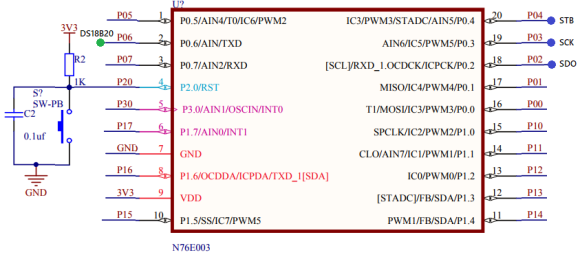## Explanation

One wire communication is detailed in these application notes from Maxim:

https://www.maximintegrated.com/en/app-notes/index.mvp/id/126

https://www.maximintegrated.com/en/app-notes/index.mvp/id/162

These notes are all that are needed for implementing the one wire communication interface for DS18B20. Please go through these notes. The codes are self-explanatory and are implemented from the code examples in these app notes.

# Decoding NEC IR Remote Protocol

IR remote controllers are used in a number of devices for remotely controlling them. Examples of these devices include TV, stereo, smart switches, projectors, etc. IR communication as such is unidirectional and in a way one wire communication. Ideally there is one transmitter and one receiver only.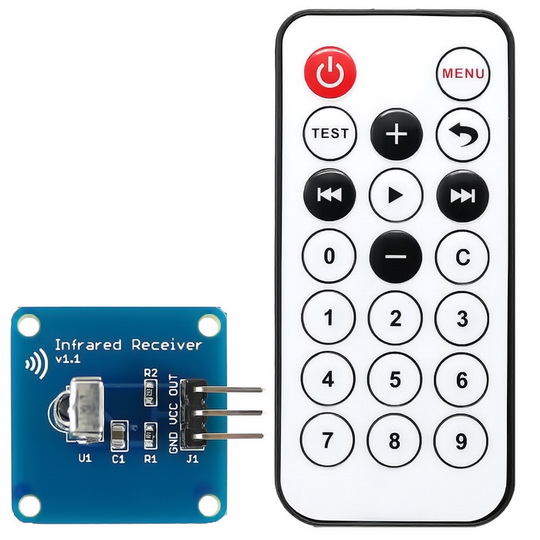Data to be sent from a remote transmitter is sent by modulating it with a carrier wave (usually between 36kHz to 40kHz). Modulation ensure safety from data corruption and long-distance transmission. An IR receiver at the receiving end receives and demodulates the sent out modulated data and outputs a stream of pulses. These pulses which vary in pulse widths/timing/position/phase carry the data information that was originally sent by the remote transmitter. How the pulses should behave is governed closely by a protocol or defined set of rules. In most micros, there is no dedicated hardware for decoding IR remote protocols. A micro that needs to decode IR remote data also doesn’t know when it will be receiving an IR data stream. Thus, a combination of external interrupt and timer is needed for decoding IR data.In this segment, we will see how we can use an external interrupt and a timer to easily decode a NEC IR remote. This same method can be applied to any IR remote controller. At this stage, I recommend that you do a study of NEC IR protocol from here. SB-Project’s website is an excellent page for info as such.

## Code

`#include "N76E003.h"`
`#include "SFR_Macro.h"`
`#include "Function_define.h"`
`#include "Common.h"`
`#include "Delay.h"`
`#include "soft_delay.h"`
`#include "LCD_2_Wire.h"`

`#define sync_high          22000`
`#define sync_low           14000`
`#define one_high            3600`
`#define one_low             2400`
`#define zero_high           1800`
`#define zero_low            1200`

`bit received;`
`unsigned char bits = 0;`
`unsigned int frames;`

`void setup(void);`
`void set_Timer_0(unsigned int value);`
`unsigned int get_Timer_0(void);`
`void erase_frames(void);`
`unsigned char decode(unsigned char start_pos, unsigned char end_pos);`
`void decode_NEC(unsigned char *addr, unsigned char *cmd);`
`void lcd_print(unsigned char x_pos, unsigned char y_pos, unsigned char value);`

`void EXTI0_ISR(void)`
`interrupt 0`
`{`
`  frames[bits] = get_Timer_0();`
`  bits++;`
`  set_TR0;`

`  if(bits >= 33)`
`  {`
`     received = 1;`
`     clr_EA;`
`     clr_TR0;`
`  }`
`  set_Timer_0(0x0000);`
`  P15 = ~P15;`
`}`

`void main(void)`
`{`
`  unsigned char address = 0x00;`
`  unsigned char command = 0x00;`

`  setup();`

`  while(1)`
`  {   `
`     if(received)`
`     {`
`       decode_NEC(&address, &command);`
`       lcd_print(13, 0, address); `
`       lcd_print(13, 1, command);`
`       delay_ms(100);`
`       erase_frames();`
`       set_EA;`
`     }`
`  };`
`}`

`void setup(void)`
`{ `
`  erase_frames();`
`  P15_PushPull_Mode;  `
`  TIMER0_MODE1_ENABLE;          `
`  set_Timer_0(0x0000);`
`  set_IT0;`
`  set_EX0;  `
`  set_EA;`

`  LCD_init();`
`  LCD_clear_home();`
`  LCD_goto(0, 0);`
`  LCD_putstr("Address:");`
`  LCD_goto(0, 1);`
`  LCD_putstr("Command:");`
`}`

`void set_Timer_0(unsigned int value)`
`{`
`  TH0 = ((value && 0xFF00) >> 8);`
`  TL0 = (value & 0x00FF);`
`}`

`unsigned int get_Timer_0(void)`
`{`
`  unsigned int value = 0x0000;`

`  value = TH0;`
`  value <<= 8;`
`  value |= TL0;`

`  return value;`
`}`

`void erase_frames(void)`
`{`
`  for(bits = 0; bits < 33; bits++)`
`  {`
`    frames[bits] = 0x0000;`
`  }`

`  set_Timer_0(0x0000);`
`  received = 0;`
`  bits = 0;`
`}`

`unsigned char decode(unsigned char start_pos, unsigned char end_pos)`
`{`
`  unsigned char value = 0;`

`  for(bits = start_pos; bits <= end_pos; bits++)`
`  {`
`    value <<= 1;`

`    if((frames[bits] >= one_low) && (frames[bits] <= one_high))`
`    {`
`      value |= 1;`
`    }`

`    else if((frames[bits] >= zero_low) && (frames[bits] <= zero_high))`
`    {`
`      value |= 0;`
`    }`

`    else if((frames[bits] >= sync_low) && (frames[bits] <= sync_high))`
`    {`
`      return 0xFF;`
`    }`
`  }`

`  return value;`
`}`

`void decode_NEC(unsigned char *addr, unsigned char *cmd)`
`{`
`  *addr = decode(2, 9);`
`  *cmd = decode(18, 25);`
`}`

`void lcd_print(unsigned char x_pos, unsigned char y_pos, unsigned char value)`
`{ `
`  LCD_goto(x_pos, y_pos);`
`  LCD_putchar((value / 100) + 0x30);`
`  LCD_goto((x_pos + 1), y_pos);`
`  LCD_putchar(((value % 10) / 10) + 0x30);`
`  LCD_goto((x_pos + 2), y_pos);`
`  LCD_putchar((value % 10) + 0x30);`
`}`

## Schematic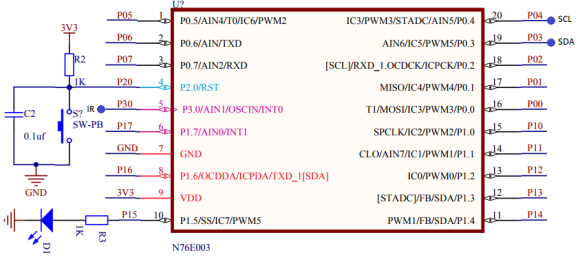## Explanation

A NEC transmission sends out 33 pulses. The first one is a sync bit and the rest 32 contain address and command info. These 32 address and command bits can be divided into 4 groups – address, command, inverted address and inverted command. The first 16 bits contain address and inverted address while the rest contain command and inverted command. The inverted signals can be used against the non-inverted ones for checking signal integrity.

We already know that IR data is received as a steam of pulses. Pulses represent sync bit or other info, ones and zeros. In case of NEC IR protocol, the pulses have variable lengths. Sync bit represented by a pulse of 9ms high and 4.5ms low – total pulse time is 13.5ms. Check the timing diagram below. Please note that in this timing diagram the blue pulses are from the transmitter’s output and the yellow ones are those received by the receiver. Clearly the pulses are inverted.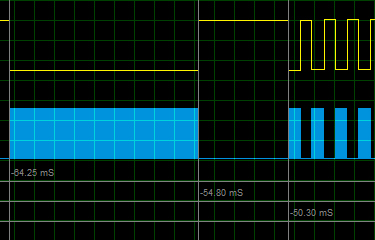Logic one is represented by a pulse of 560µs high time and 2.25ms of low time – total pulse time is 2.81ms. Likewise, logic zero is represented by a pulse of 560µs high time and 1.12ms of low time – total pulse time is 1.68ms.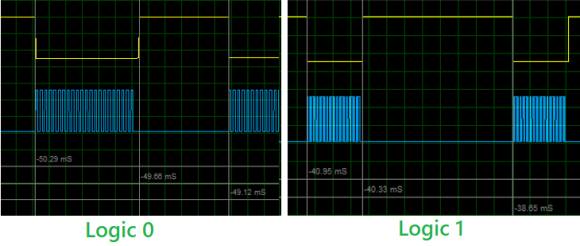These timings can vary slightly about 15 – 25% due to a number of factors like medium, temperature, etc. Therefore, we can assume the following timings:Now as per timing info and timing diagram we have to detect falling edges and time how long it takes to detect another falling edge. In this way, we can time the received pulses and use the pulse time info to decode a received signal.

In the demo, I used external interrupt channel EXTI0 and Timer 0. The system clock frequency is set to 16MHz with HIRC. Timer 0 is set in Mode 1 and its counter is rest to 0 count. EXTI0 is set to detect falling edges. Note that the timer is set but not started.

`TIMER0_MODE1_ENABLE;          `
`set_Timer_0(0x0000);`
`set_IT0;`
`set_EX0;  `
`set_EA;`

Also note that each tick of Timer 0 here is about:Based on this tick info, we can deduce the maximum and minimum pulse durations as like:

`#define sync_high          22000 // 22000 × 0.75ms = 16.5ms`
`#define sync_low           14000 // 14000 × 0.75ms = 10.5ms`
`#define one_high            3600 // 3600 × 0.75ms = 2.7ms`
`#define one_low             2400 // 2400 × 0.75ms = 1.8ms`
`#define zero_high           1800 // 1800 × 0.75ms = 1.35ms`
`#define zero_low            1200 // 1200 × 0.75ms = 0.9ms`

Now when an IR transmission is received, an interrupt will be triggered. Therefore, in the ISR, we immediately capture the timer’s tick count and reset it. When all 33 pulses have been received this way, we temporarily halt all interrupts by disabling the global interrupt and stop the timer in order to decode the received signal. P15 is also toggled to demonstrate reception.

`void EXTI0_ISR(void)`
`interrupt 0`
`{`
`  frames[bits] = get_Timer_0();`
`  bits++;`
`  set_TR0;`

`  if(bits >= 33)`
`  {`
`     received = 1;`
`     clr_EA;`
`     clr_TR0;`
`  }`
`  set_Timer_0(0x0000);`
`  P15 = ~P15;`
`}`

Now the signal is decoded in the decode function. This function scans a fixed length of time frames for sync, ones and zeros and return the value obtained by scanning the time info of the pulses. In this way, a received signal is decoded.

`unsigned char decode(unsigned char start_pos, unsigned char end_pos)`
`{`
`  unsigned char value = 0;`

`  for(bits = start_pos; bits <= end_pos; bits++)`
`  {`
`    value <<= 1;`

`    if((frames[bits] >= one_low) && (frames[bits] <= one_high))`
`    {`
`      value |= 1;`
`    }`

`    else if((frames[bits] >= zero_low) && (frames[bits] <= zero_high))`
`    {`
`      value |= 0;`
`    }`

`    else if((frames[bits] >= sync_low) && (frames[bits] <= sync_high))`
`    {`
`      return 0xFF;`
`    }`
`  }`

`  return value;`
`}`

In the main loop, the decode info are displayed on a text LCD.

## Demo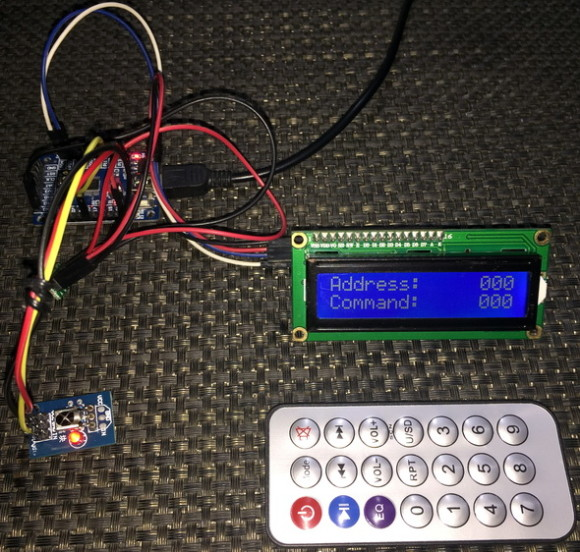# Epilogue

After going through all these topics and exploring the N76E003 to the finest details, I have to say that just like flagship killer cell phones, this is a flagship killer 8-bit microcontroller. In terms of price vs feature ratio, it is a winner. I’m truly impressed by its performance and hardware features. It offers something like an 8051 but with more advanced modern features. Old-school 8051 users will surely love to play with it.

Every day we talk about software piracy and hacking but little effort is done to prevent them. Students, hobbyists and low-profile business houses can’t afford to use the expensive Keil/IAR compiler. They often go for pirated versions of these software and this is a very wrong path to follow. Like I said in my first post on N76E003, Nuvoton has rooms for making its devices more popular by investing on a compiler of its own or at least start doing something with free Eclipse-IDE.

All files and code examples related to Keil compiler can be downloaded from here.

All files and code examples related to IAR compiler can be downloaded from here.

Happy coding.

Author: Shawon M. Shahryiar

06.08.2018

### Related Posts

• Tarun PatelHello Sir, I have a question about #define one_low / one_high / zero_high / zero_low value of timings are different form caclulated timing in above chart. In chart for one low 2.2ms and in #define its 1.8ms why is it like that?

•because we have to take some environmental factors like air temperature, medium, etc. into account….

• Shweta ThoratHello Sir,

My name is Shweta. I have started working on Nuvoton controllers, I want to read temperature using 3 Channel Seven seg- Display. controller have inbuilt 12 bit ADC . I have written the below code but its not working can please check. (N76E003AT20)

#include “N76E003.h”
#include “Function_Define.h”
#include “SFR_Macro.h”
#include “common.h”
#include “Delay.h”
#include “math.h”
#define bit_to_voltage_ratio 0.001220703125 // 5.0V divided by 4096 For 12-Bit ADC

#define set_P17DIDS AINDIDS |= SET_BIT0
#define AIN0 P17
#define Seg_a P10
#define Seg_b P11
#define Seg_c P12
#define Seg_d P13
#define Seg_e P14
#define Seg_f P15
#define Seg_g P16
#define Seg_h P30

int adc_val, R1 = 0, count = 0, fonts;
void sw_delay(int ms)
{
int a, b;
for(a=0; a<1000; a++){
for(b=0; b<ms; b++);
}}
void setup(void)
{
P10_Input_Mode;
P11_Input_Mode;
P12_Input_Mode;
P13_Input_Mode;
P14_Input_Mode;
P15_Input_Mode;
P16_Input_Mode;
P17_Input_Mode;
}

int demo(void)
{
// char ptr[]={0x40, 0x79, 0x24, 0x30, 0x19, 0x12, 0x02, 0x78, 0x00, 0x10};
// char i ;
// while(1)
// {
// for(i=0; i<99; i++)
// {
// P17_Input_Mode;
// P1 = ptr[i];
// //sw_delay(100);
// }
// } //(0b100000, 0b1111001, 0b0010010, 0b0110000, 0b0011001, 0b0010010, 0b0000010, 0b1110000, 0b0000000, 0b0010000);

setup();
sw_delay(1000);
Seg_a = 0;

Seg_b = 0;

Seg_c = 0;

Seg_d = 0;

Seg_e = 0;

Seg_f = 0;

Seg_g = 1;

Seg_h = 0;

while(1)
{
switch (count)

{
case 0:
Seg_a = 0;

Seg_b = 0;

Seg_c = 0;

Seg_d = 0;

Seg_e = 0;

Seg_f = 0;

Seg_g = 1;

Seg_h = 0;

sw_delay(1000);

break ;

case 1:
Seg_a = 1;
Seg_b = 0;

Seg_c = 0;
Seg_d = 1;

Seg_e = 1;

Seg_f = 1;

Seg_g = 1;
Seg_h = 0;
sw_delay(1000);

break;
case 2:

Seg_a = 0;

Seg_b = 0;
Seg_c = 1;
Seg_d = 0;

Seg_e = 0;
Seg_f = 1;
Seg_g = 0;

Seg_h = 0;
sw_delay(1000);

break;
case 3:

Seg_a = 0;

Seg_b = 0;

Seg_c = 0;

Seg_d = 0;
Seg_e = 1;
Seg_f = 1;
Seg_g = 0;
Seg_h = 0;
sw_delay(1000);

break;
case 4:
Seg_a = 1;
Seg_b = 0;

Seg_c = 0;
Seg_d = 1;
Seg_e = 1;
Seg_f = 0;

Seg_g = 0;
Seg_h = 0;
sw_delay(1000);

break;
case 5:

Seg_a = 0;
Seg_b = 1;
Seg_c = 0;

Seg_d = 0;
Seg_e = 1;
Seg_f = 0;

Seg_g = 0;

Seg_h = 0;

sw_delay(1000);

break;
case 6:

Seg_a = 0;
Seg_b = 1;
Seg_c = 0;

Seg_d = 0;

Seg_e = 0;

Seg_f = 0;

Seg_g = 0;

Seg_h = 0;
sw_delay(1000);

break;
case 7:

Seg_a = 0;

Seg_b = 0;

Seg_c = 0;
Seg_d = 1;

Seg_e = 1;

Seg_f = 1;

Seg_g = 1;
Seg_h = 0;
sw_delay(1000);

break;
case 8:

Seg_a = 0;

Seg_b = 0;

Seg_c = 0;

Seg_d = 0;

Seg_e = 0;

Seg_f = 0;

Seg_g = 0;
Seg_h = 0;

break ;
case 9:

sw_delay(1000);
Seg_a = 0;

Seg_b = 0;

Seg_c = 0;

Seg_d = 0;
Seg_e = 1;
Seg_f = 0;

Seg_g = 0;
Seg_h = 0;
sw_delay(1000);

break;
break;
}
if (count 0) && (value 9) && (value <= 99))
{
send_data((2 + segment), 0);
ch = (value / 10);
send_data((1 + segment), fonts[1 + ch]);
ch = (value % 10);
send_data(segment, fonts[1 + ch]);
}
else
{
send_data((2 + segment), 0);
send_data((1 + segment), 0);
ch = (value % 10);
send_data(segment, fonts[1 + ch]);
}
}

void main (void)
{
demo(); //For GPIO1 output, Find in "Function_define.h" – "GPIO INIT"
setup();
display_data();
while(1);
{
// Each time ADC start trig signal
clr_GPIO1;
sw_delay(1000);
set_GPIO1;
R1 = R1*1000; //Resistance in ohm
}
}

• Elektronik ExpertsLED Segment pins defined as input, first of all make LED Segments and Common pins output

• Akshita GandhiI try to connect DHT22..and send data over UART but there is an error

void main(void)
{
char DHT_ASCII = “0000000000000000000000000000000000000001\0”;
int DHT_Status = 0;
LED_Init;

Serial_TX(“start \r\n”);

TI = 1; // prinft function is used.
printf(“+————————————-+ \n”);
printf(“| Temperature Sensor Code | \n”);
printf(“+————————————-+ \n”);

while(1)
{

LED_OFF;
Timer0_Delay1ms(500);
LED_ON;
Timer0_Delay1ms(500);

DHT22_start();
dht22_response();

if (DHT_Status==DHTLIB_OK) Serial_TX(DHT_ASCII);
}

}

/////////////////////Serial//////////////////

void Serial_TX(char *str)
{
unsigned int j;
P06_Quasi_Mode;
P07_Quasi_Mode;
P10_Quasi_Mode;
P11_Quasi_Mode;

SCON = 0x50; //UART0 Mode1,REN=1,TI=1
TMOD |= 0x20; //Timer1 Mode1,
set_SMOD;
set_T1M;
clr_BRCK; //Serial port 0 baud rate clock source = Timer1
TH1 = 152; //9600 baud rate
set_TR1; //Start timer1

for(j=0;str[j]!=0;j++)
{
SBUF=str[j];
while(TI==0)
{}
TI=0;
}

Timer0_Delay1ms(500);
}

/////////////////////end of serial//////////////////

/////////////////////Delay function//////////////////
void delay_us(unsigned int value)
{
value = (value / osc_scalar);

while(value > 0)
{
nop;
value–;
}
}

void delay_ms(unsigned int value)
{
while(value > 0)
{
–value;
delay_us(1000);
}
}

/////////////////////END//////////////////

/////////////////////DHT22 function//////////////////
void DHT22_start(void)
{
DHT22_pin_OUT;
DHT22 = 0;
delay_ms(12);
DHT22 = 1;
delay_us(30);
DHT22_pin_IN;
}

void dht22_response(void)
{
DHT22_pin_IN;
delay_us(40);

if(!DHT22)
{
delay_us(80);

if(DHT22)
{
Serial_TX(“sensor response\r\n”);
}else
{
Serial_TX(“sensor not response\r\n”);
}
}
delay_us(40);
//while(DHT22);
}

{
unsigned char i ;
DHT22_pin_IN;
// EMPTY BUFFER
for (i = 0; i < 40; i++) buf[i] = '0'; buf=0;

for (i = 40; i != 0; i–)
{

while(DHT22 == 0);

delay_us(40);

if(DHT22)
{
buf[i] = '1';
}
while(DHT22 == 1);
}

DHT22_pin_OUT;
DHT22 == 1;

return DHTLIB_OK;
}

•Sensor is a necessary means for the robot to complete the feeling. Through the sensory function of the sensor, the relevant characteristics of the robot or the characteristics of related objects are transformed into the information required by the robot to perform a function.

•I tried using your example and porting it to my controller but was not successful.

•Hi, i am new to Nuvoton controllers. i purchased MS51FB9AE IC’s and N76E003 Development board, can i replace the N76E003 IC with MS51FB9AE IC and use same board and same examples.

• Chetan GautamHi Shawon Shahryiar,
I am trying to interface DS18B20 with MS51FB9AE nuvoton microcontroller . I am using your project only , in which i have just changed the pin , i am using P17 . I have made this pin in Open Drain Mode and pull up with 4.7k resistor . I am operating it with 3.3 V supply . But it is not working . Any suggestion you can give

•May be the clock speeds are different….

• Sudarshan SrinivasanI have used N76E003 IC only but facing same problem. I have defined FOSC as 160000 as suggested in its sample codes. Can you tell what clock speed u have defined in ur project? Or any other initialization have to be done?

• ramgopali am trying load the ldrom with bootloader code so that aprom could be prgrammed through serial port.
i am not able to find this info.
will you kindly indicate a method to have the ldrom programmed with bootloader.
i am retired person and programming microcontrollers is my habit.
thanks
ramgopal

•Hi,
Can I set I2C address for N76E003 using external hardware such as dip-switch. I tried datasheet reading but found no way to set the I2C address for the chip externally without hard-coding in code.
Ngoc

• ARJUN SURENDRAN@Shawon Shahryiar.
Can you provide me the DS1307 I2C code using Nuvuton ms51fb9ae controller??
I tried using your example and porting it to my controller but was not successful.
Any help and support is appreciated.

• Pravallika Kolisetty@Shawon Shahryiar: Thank you for explaining very clearly …
I am writing a code for DHT11 sensor on nuvoton MS51FB9AE controller.. Currently i am facing issue is that i am not getting acknowledgment from DHT11 sensor… Looks like the delay code i implemented might be having issues…
I am referring to your program under section ‘One Wire (OW) Communication – Interfacing DHT11’ … In your program the delay_us() and delay_ms() functions are called…. Looks like the definition for these functions might be implemented in soft_delay.h file…
Can i get the source code of soft_delay.h and soft_delay.c?

Also can you share the path of all the programs from where i can download?

• Pravallika KolisettyGot the source code path ‘https://drive.google.com/open?id=1rLNJWHODC2I4-iQGLyWpdU47Md1WZ0aP’

Thank you

• Jimy_BDHi Sir
I have some problem, with DHT sensor. i am use timer 1 interrupt for multiplex 4 digit 7 segment display. without interrupt sensor reading is working perfectly. but after enable interrupt, DHT reading not properly. any sulution sir ?

it’s possible with Pulse Width Capture or using other timer ?

•I think there is pin conflict in your project….

• Niels PedersenHi , thank you for the tutorial and thank for your time to answer questions.

I tried the last tutorial “NEC IR to LCD”. and spent a lot of time troubleshooting until I got the idea to watch the accompanying movie.

As I see it, the buttons obviously have the same “address”. BUT some of the buttons also have the same “Command”.

In theory, it may make sense if the response depends on the condition / mode of the equipment. But I do not see any point in having the “4” and “5” keys to have the same “Command”.

I have tried with some 16 and 20 button remotes and get the same values as in your movie.

But I have also tried a remote control for some LED candles. Here I could also get some of the same values – “Address / Command”. But my remote controls can not control the candles with the same values ????

So I want to ask: Are you sure the program is decoding the keys correctly? I was wondering if a bit could be lost, but then it would not be able to give the values 202,205,209 etc.

•Yes decoding correctly…. If a bit is lost somehow then the received data-command and their inverted forms would be different and would result in error….

• Milan PatelSir i send nec data and it received perfect with wire comminucation,but i am unable to wireless communication using ir led.

So please suggest circuit diagram or any suggestions.

Thankx

• Milan PatelSir can you share code for nec ir transmission using n76e003.

•What did you mean by that? It has already been shared….

• Milan Pateli want to make nec ir remote. so require that example.

•Dear Shawon Shahryiar
Thanks for this great tutorial, its very clear and impressive

•Hello sir,

• Nilesh PatleI Folks,

I am trying to interface the MLX90614 Temperature sensor with N76E003. But when I read the data through I2C getting 255 all time.

I would request to all kindly find the my bellow code and please help to solve the problem:

#include “N76E003.h”
#include “SFR_Macro.h”
#include “Function_define.h”
#include “Common.h”
#include “Delay.h”
//#include “MLX90614.h”
#include “I2C.h”

// Set the UART global flags
unsigned char temp _at_ 0x08;
unsigned char idata itemp _at_ 0x80;
unsigned char xdata xtemp _at_ 0x80;

unsigned int I2C_W = 0;
unsigned int I2C_R = 1;

// Global Functions for MLX90614 temperature Sensor
// RAM
#define MLX90614_RAWIR1 0x04
#define MLX90614_RAWIR2 0x05
#define MLX90614_TA 0x06
unsigned int MLX90614_TOBJ1 = 0x07;
//MLX90614_TOBJ1 = 0x07;
#define MLX90614_TOBJ2 0x08

// Initilize the Temperature Sensor functions
void MLX90614_init(){
I2C_init();
// printf(“\n start I2C”);
}

}

float temperature;
printf(“Temp reg %f \n”, temperature);
temperature *= .02;
printf(“Temp 0.02 %f \n”, temperature);
temperature -= 273.15;
printf(“Temp Finals %f \n”, temperature);
return temperature;
}

unsigned int read16(unsigned int a) {
unsigned int ret = 0;
unsigned int Eight = 8;
unsigned int ret1 = 0;
I2C_start(); // Send the start bit
I2C_write(a); // Send the dataa

I2C_start();
printf(“First ret: %d \n”, ret);
ret1 = ret1 << Eight;
ret = ret | ret1;
I2C_stop();
printf("Second ret: %d\n", ret1);
return ret;
}

/*==========================================================================*/
void main (void)
{
InitialUART0_Timer3(115200);
TI = 1; // Important, use prinft function must set TI=1;
printf("\n________*____Main*_________");
MLX90614_init();
while(1)
{
Timer0_Delay1ms(100);
}
}

•Hello sir,
I am trying to interface si7021 with n76e003,I do follow same i2c library as you mention in this article and my furthur code is as below. I am not be able to get answer can you please guide me where I need to change my code.

I2C_init();
InitialUART0_Timer3(115200);
TI=1;

I2C_start();
I2C_write(0x40);
I2C_write(0xF3);
I2C_stop();
delay_1ms_T1(180);

while(1)
{

I2C_write(0x40);
I2C_stop();
value1 = value1<<8;
value3 = value1|value2;
temp=(175.72*value3)/65536;
temp=temp-46.85;

printf("\n Value1 is:%d",value1);
delay_1ms_T1(2000);
printf("\n Value2 is:%d",value2);
delay_1ms_T1(2000);
printf("\n Temp is:%d",temp);
delay_1ms_T1(2000);
value1 = 0;
}

• amar axrHOW TO INTERFACE LCD16X2 WITH NUVOTON MS51FB9AE IC ?
my lcd pins connection to MS51FB9AE is as follows:

RS – P04

EN – P03

D4 – P01

D3 – P00

D2 – P10

D1 – P11

I want to run it in 4bit mode

•Have you seen the 4-bit LCD example I showed in the article? You just have to change the pins there.

• Shankaranarayanan P VHi sir,
I am trying to interface n76e003 with vcnl4200 via I2C, everytime I write to the slave address, and read the I2STAT register I am reading it as f8. I looked up in the datasheet for this status code, and it mentions it as I2C bus released. What could be the problem here? The start command is issued correctly, the problem occurs when i try to write.

#define VCNL4200_DeviceID_REG 0x0e

void VCNL_init(void)
{
I2C_init();
I2C_start();
if(I2STAT == 0x08)
{
printf(“\n start issue”);
}
else
{
printf(“\n start not issue”);
I2C_init();
I2C_start();
}
//I2C_write(VCNL4200_DeviceID_REG);
if(I2STAT == 0x18)
{
printf(“\nSla+w has been issued”);
printf(“\n I2STAT is 0%0x”,I2STAT);
}
else
{
printf(“\n sla+w not issued”);
printf(“\n I2STAT is %x”,I2STAT);
}
I2C_start();
I2C_stop();
printf(“\nValue is 0x%0x”,value);
}
Kindly help me on this issue.

•try to get rid of the codes like the printfs in between your I2C code….

• Shankaranarayanan P VHi sir,
I removed the printf statements, but still it was not working properly. After which I changed the SDA, SCL pins to Quasi Mode. After which I am able to read data. But still the data seems to be wrong. I am trying to read the device Id of the IC. The result should be 0x58 on low byte and 0x10 on high byte. But I am getting it as 0x6060. Kindly check whether I have written the code correctly.

#define VCNL4200_DeviceID_REG 0x0E
#define I2C_CLOCK 27 //Fclk = Fsys / (4*(prescalar + 1))
void VCNL_init(void)
{
UINT8 lb=0;
UINT8 hb=0;
UINT8 status1=0;
UINT8 status2=0;
Set_All_GPIO_Quasi_Mode;
//P14_OpenDrain_Mode;
I2C_init();
I2C_start();
if(I2STAT != 0x08)printf(“\n St not ok”); /*I2STAT 0x08 -start transmit success*/
I2C_write(VCNL_WR);
if(I2STAT != 0x18)printf(“\n w not ok1”); /*I2STAT 0x18 -start write success*/
I2C_write(VCNL4200_DeviceID_REG);
if(I2STAT != 0x28)printf(“\n w not ok2,I2STAT is %x”,I2STAT); /*I2STAT 0x28 -data transmit success*/
I2C_start();
if(I2STAT != 0x08)printf(“\n St not ok”); /*I2STAT 0x08 -start transmit success*/
I2C_write(VCNL_RD);
if(I2STAT != 0x40)printf(“\n w not ok1, I2STAT is 0x%x”,I2STAT); /*I2STAT 0x18 -start write success*/
I2C_stop();
printf(“\n low Byte is 0x%x, Low Byte is 0x%x”,lb,hb);
}

I am getting High Byte as 0x6060 and Low byte as 0x00. The in-between I2C status are coming correctly.

•hello,i am ratan,i want to do working with N76E003 board.
But the problem is how to load the hex file in N76E003 board ?
which software i will use for burning, and programmer i will use ?

can any body please tell me

•• Ashutosh KedareCan we use NuMicro controllers in products of our company . As we know this controllers are totally new can we really trust on them for there performance and all ?

•Of course without a doubt as there are lot devices and gadgets based on NuMicros….

•hi. i am miths,was working with 76e003.
i) i need to find sample code for Brown out detection of 76e003
ii) can i interface nrfl01 or LoRa module RA-02 with 76e003.

I need its code. can any body please provide these two codes?

•Nice tutorial about Nuvoton controllers. One thing I really got out of it that made a difference is the part about your explanation about hardware and source code. It’s very helpful to learn the nuvoton. Thank you for sharing the details and code.

Can you share the details of I2C master-slave communication between to nuvoton controllers or maybe add that into your post?
I have check the code from below link but it wont work..
https://github.com/OpenNuvoton/N76E003-BSP/blob/master/Sample_Code/I2C_Master-Slave/I2C_Slave/Code/I2C_S.c

Thank You!

•That’s in plan for future posts….

•Hello sir,
When i test BH1750 sensor with nuvoton(via I2C),only get an integer value 255 as response,why??
anything that i want to modify ?
thanks..

#define regular_I2C_pins 0

#define alternate_I2C_pins 1

#define regular_I2C_GPIOs() do{P13_OpenDrain_Mode; P14_OpenDrain_Mode; clr_I2CPX;}while(0)

#define alternative_I2C_GPIOs() do{P02_OpenDrain_Mode; P16_OpenDrain_Mode; set_I2CPX;}while(0)

#define I2C_GPIO_Init(mode) do{if(mode != 0){alternative_I2C_GPIOs();}else{regular_I2C_GPIOs();}}while(0)

#define I2C_CLOCK 0x27 //Fclk = Fsys / (4*(prescalar + 1))

#define I2C_ACK 0

#define I2C_NACK 1

#define timeout_count 1000

void I2C_init(void);

void I2C_start(void);

void I2C_stop(void);

void I2C_write(unsigned char value);
void GetLightIntensity(void);

#include “N76E003.h”

#include “SFR_Macro.h”

#include “Function_define.h”

#include “Common.h”

#include “Delay.h”

void I2C_init(void)

{

regular_I2C_GPIOs();

I2CLK = I2C_CLOCK;

set_I2CEN;

}

void I2C_start(void)

{

set_STA;

clr_SI;

while (!SI);

}

void I2C_stop(void)

{

clr_SI;

set_STO;

while((STO == 1))

{

};

}

{
unsigned char value = 0x00;
if(ack_mode == I2C_NACK)
{
set_AA;
clr_SI;
while((SI == 0));

value = I2DAT;

}

if(ack_mode == I2C_NACK)

{

clr_AA;

clr_SI;

while((SI == 0) );
value = I2DAT;
}

return value;

}

void I2C_write(unsigned char value)

{

I2DAT = value;

clr_STA;

clr_SI;

while((SI == 0))

{

};

}
void GetLightIntensity(void)
{
uint16_t Value = 0;

I2C_start();
I2C_write(0x23);
Value <<= 8;
I2C_stop();

printf("%d",Value);

}

void main()
{
int cp;
InitialUART0_Timer3(9600);
I2C_init();
I2C_start();
I2C_write(0x23);
I2C_write(0x10);
I2C_stop();
Timer0_Delay1ms(180);
while(1)
{
GetLightIntensity();

}

}

•I2C bus pins pulled up?

•sir..i am not add any pull up resistor externally for both SDA and SCL line.but i think GY30(bh1750) light intensity sensor module has inbuilt two pull up resistor .

•Okay…. the code should be like this:

``` void BH1750_init() { delay_ms(100); BH1750_write(power_down); } ```

``` void BH1750_write(unsigned char cmd) { I2C_start(); I2C_write(BH1750_address_w); I2C_write(cmd); I2C_stop(); } unsigned long BH1750_read_word() { register unsigned long value = 0; unsigned char lb = 0; unsigned char hb = 0; I2C_start(); I2C_write(BH1750_address_r); hb = I2C_read(1); lb = I2C_read(0); I2C_stop(); value = make16(hb, lb); return value; } ```

```unsigned long get_lux_value(unsigned char mode, unsigned long delay_time) { register unsigned long lux_value = 0; BH1750_write(power_up); BH1750_write(mode); lux_value = BH1750_read_word(); delay_ms(delay_time); BH1750_write(power_down); return lux_value; } ```

• vamsi krishna*** ERROR L121: IMPROPER FIXUP
MODULE: .\Objects\main.obj (MAIN)
SEGMENT: ?PR?MAIN?MAIN
OFFSET: 0000H

could you tell me why im getting this error i tried to make my own libraries

•What did you do? Did you follow the procedure as depicted in the project setup videos? Which compiler are you using?

• vamsi krishna*** ERROR L121: IMPROPER FIXUP
MODULE: .\Objects\main.obj (MAIN)
SEGMENT: ?PR?MAIN?MAIN
OFFSET: 0000H

could you tell me why im getting this errorHi , thank you for the tutorial its really helpful.

I have done some programming using N76E003 minimal development board and i was using NU-LINK as online programming to flash device.

Now I want to run this controller standalone with newly created software embedded in it, I mean without connecting NULINK programmer (SWD) interface.

for this , first I have to program the software (via Online NuLink programming )and then disconnect the Programmer, provide external power supply and it should run. But it’s not happening. Is this the way ?

Can you suggest what’s wrong happening, should I do this on the breadboard first? If yes i am new to hardware , just give me some kickstart for hardware schematics !! am I giving the wrong power?

•Hi,

I would like to get pulse data via “INT1/ADC_CH0/P1.7” in N76E003.
But i am beginner in this part. Can anyone give me any advice how to approach or sample code?
I am even now confusing between PIN interrupt in External Interrupt too.

Best regards,
Ryan.

• Sudhanshu AwasthiHi,
Can you send me the N76E003 interfacing with Bluetooth module HC-05

•I showed how to use N76E003’s UART…. You can use that example to interface GPS, serial Bluetooth, communication modules, etc….

• NandhakumarCan you help me in BOD interrupt? I am try to get values stored when BOD occures.

• Chan Li ChouHello, I’m Chan Li form the Marketing Communication department of Nuvoton. We really enjoy your in-depth articles of Nuvoton N76E003. In 2019 we launched the upgraded version of N76E003, MS51 series. The pin assignment is completely the same. We are wondering if you would be interested in giving it a trial. We can send you a few product samples and development boards.

Best,
Chan Li Chou

•Thanks for appreciating my article…. I would definitely love to try new Nuvoton chips….

•How much minimum time we can achieve in PIN toggle for N76E003?
internal clock is 20MHz. Is there is any internal clock divider…? The chip says 1T.

•Firstly internal clock is not more than 16MHz (HIRC)…. If you use 20MHz, it will be overclocked…. There is a clock divider present that can divide selected clock source…. I have written the following three functions to deal with the clock system:

void set_clock_source(unsigned char clock_source);
void disable_clock_source(unsigned char clock_source);
void set_clock_division_factor(unsigned char value);

Indeed it has a 1T 8051 core….

•I had tried with it but the port pins on/off delay is showing 390ns in slow slew rate. How can we go for fast slew rate? I had tried with Slew Rate register but its making no difference. Please help.

•Those conditions are for special cases like SPI/UART…. When using ordinary GPIOs, these characteristics won’t be visible….

• Amir ShaikhVery nice tutorial on Nuvoton controllers.
Thank you for making it too easy to understand and sharing the codes.

Have you interfaced WS2811/UCS1903 RGB Pixel drive with Nuvoton 76E003?
Can you share the code with us?

I had tried with it but the port pins on/off delay is showing 390ns in slow slew rate. How can we go for fast slew rate? I had tried with Slew Rate register but its making no difference.